# RD Sharma Solutions For Class 7 Maths Chapter - 7 Algebraic Expressions

In order to excel in exams, students can view and download the PDF of RD Sharma Solutions for Class 7 Maths Chapter 7 Algebraic Expressions. RD Sharma Solutions are useful for students as it helps them in scoring high marks in the examination. These solutions are prepared by subject matter experts at BYJU’S, describing the complete method of solving problems.

Chapter 7, Algebraic Expressions, contains four exercises. RD Sharma Solutions for Class 7 given here includes the answers to all the questions present in these exercises. Let us have a look at some of the concepts that are being discussed in this chapter.

• Definition and meaning of algebraic expressions
• Types of algebraic expressions
• Definitions of factors and coefficients
• Like and unlike terms
• Finding the value of algebraic expressions
• Operations on algebraic expressions
• Addition of positive like terms
• Addition of negative like terms
• Addition of positive and negative like terms
• Addition of algebraic expressions with like and unlike terms
• Subtraction of algebraic expressions
• The use of grouping symbols in writing algebraic expressions
• Removal of brackets

## Download the PDF of RD Sharma Solutions For Class 7 Maths Chapter 7 Algebraic Expressions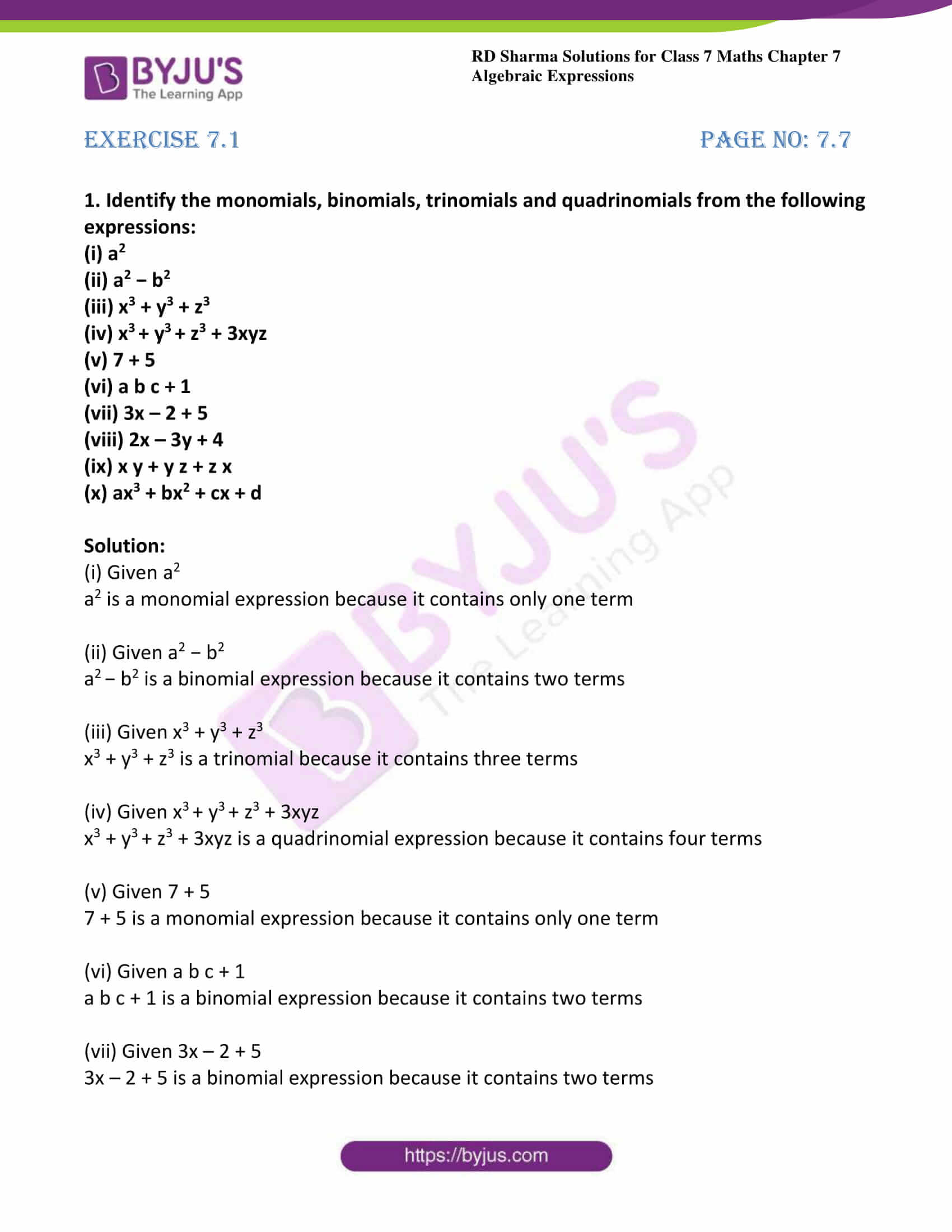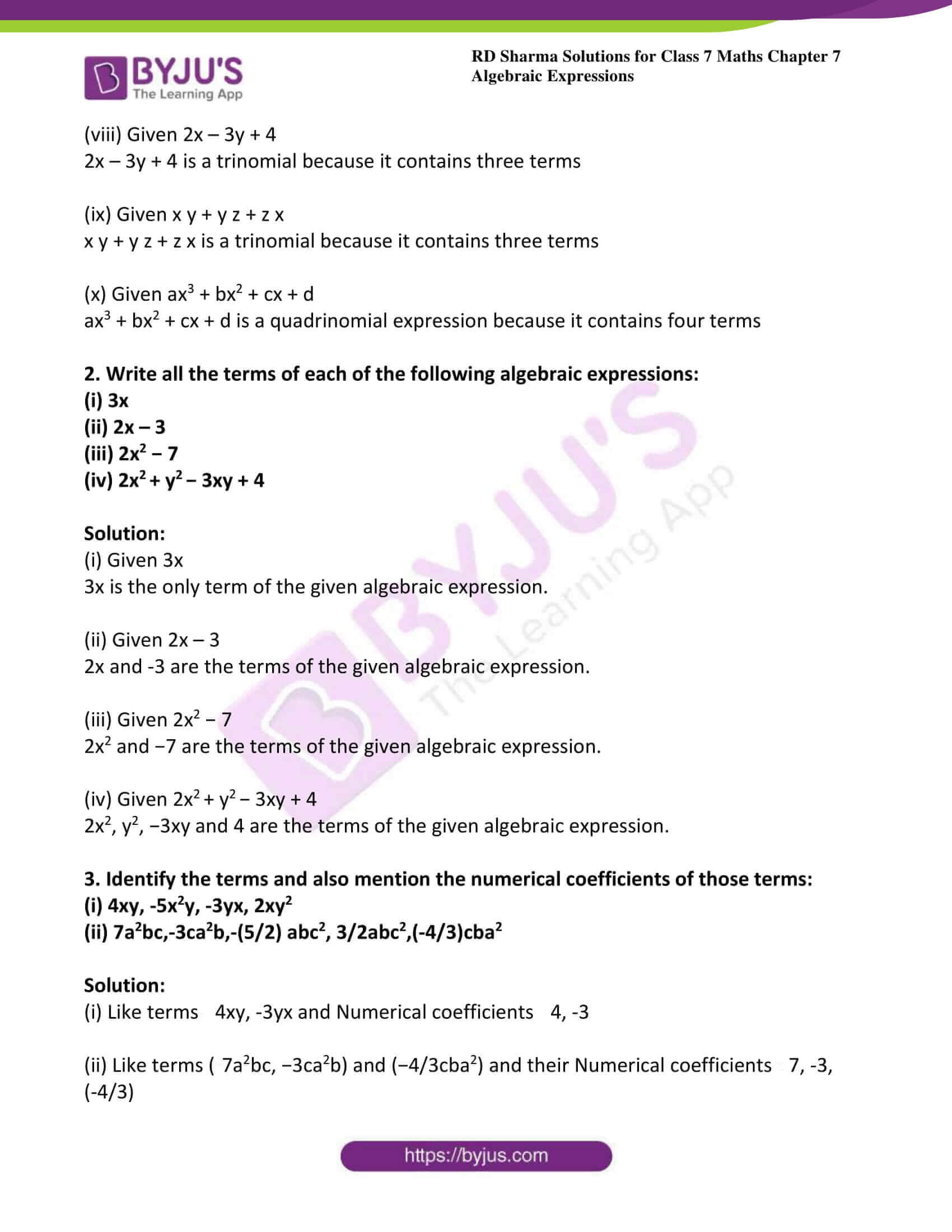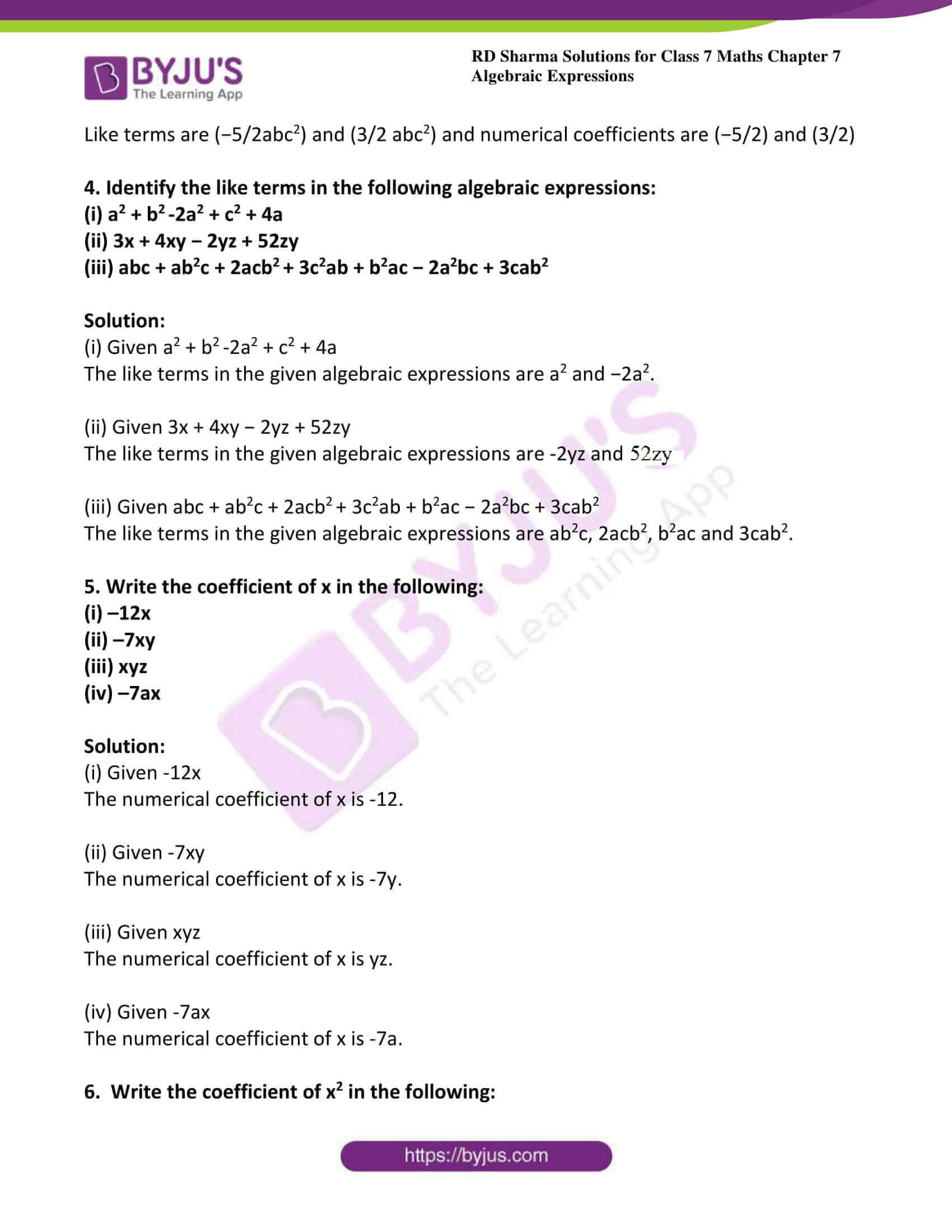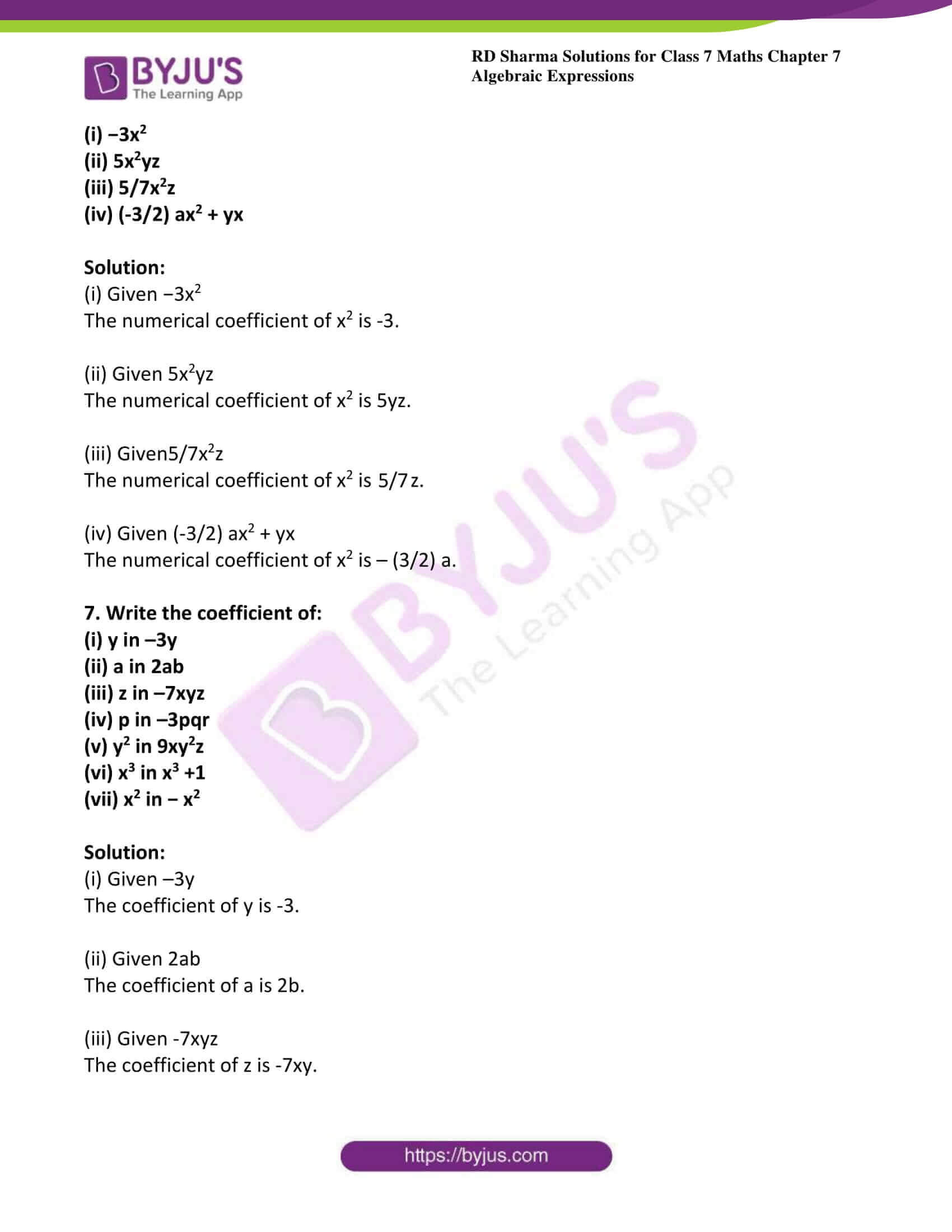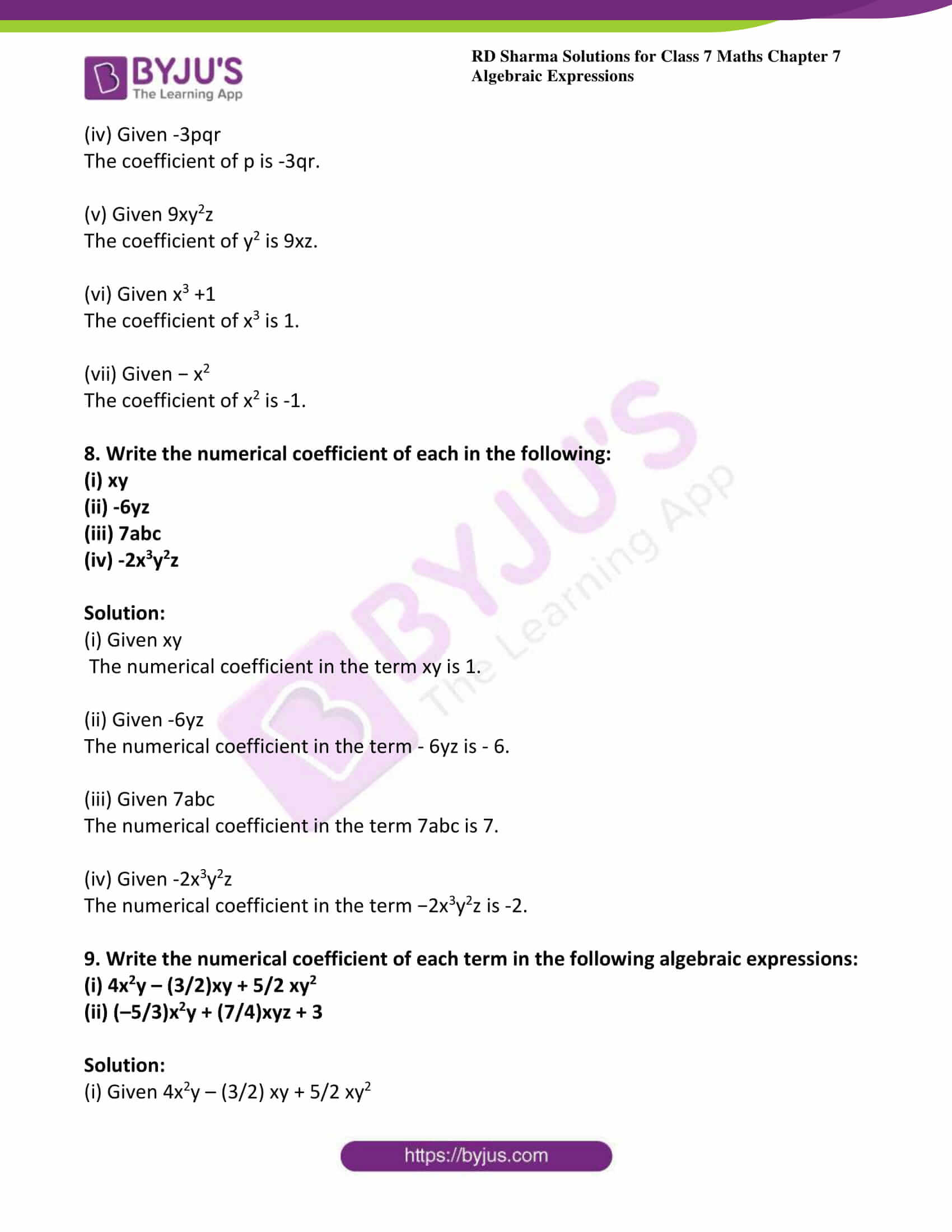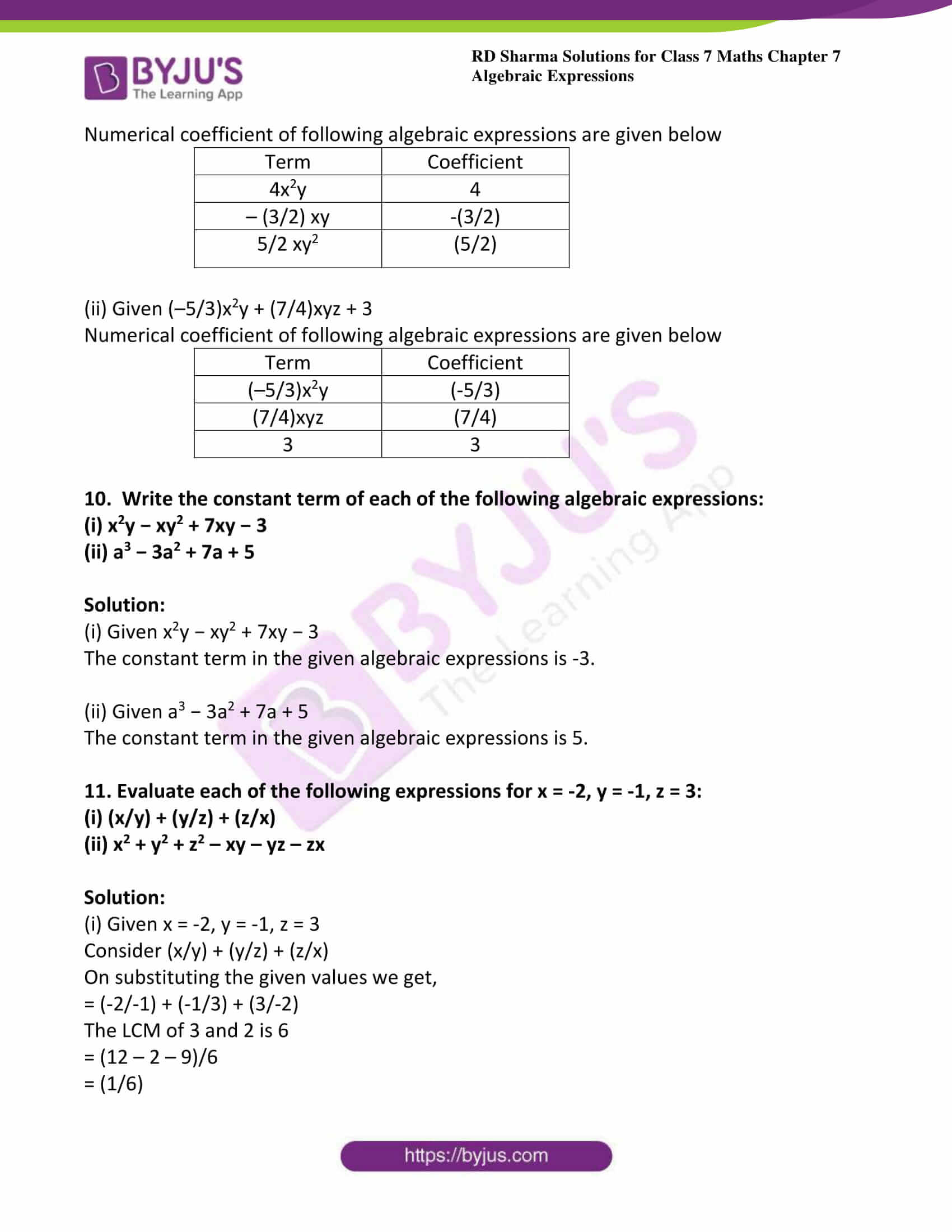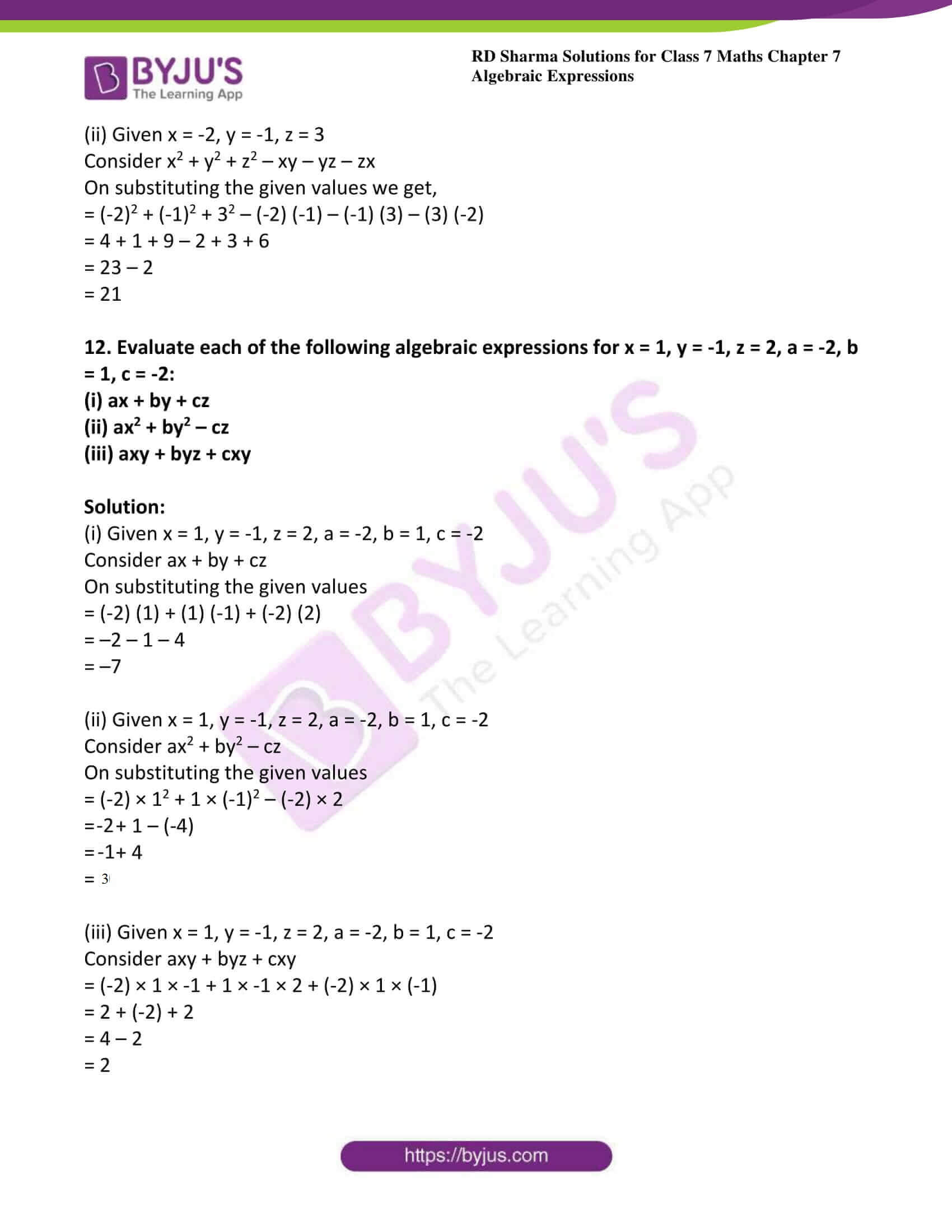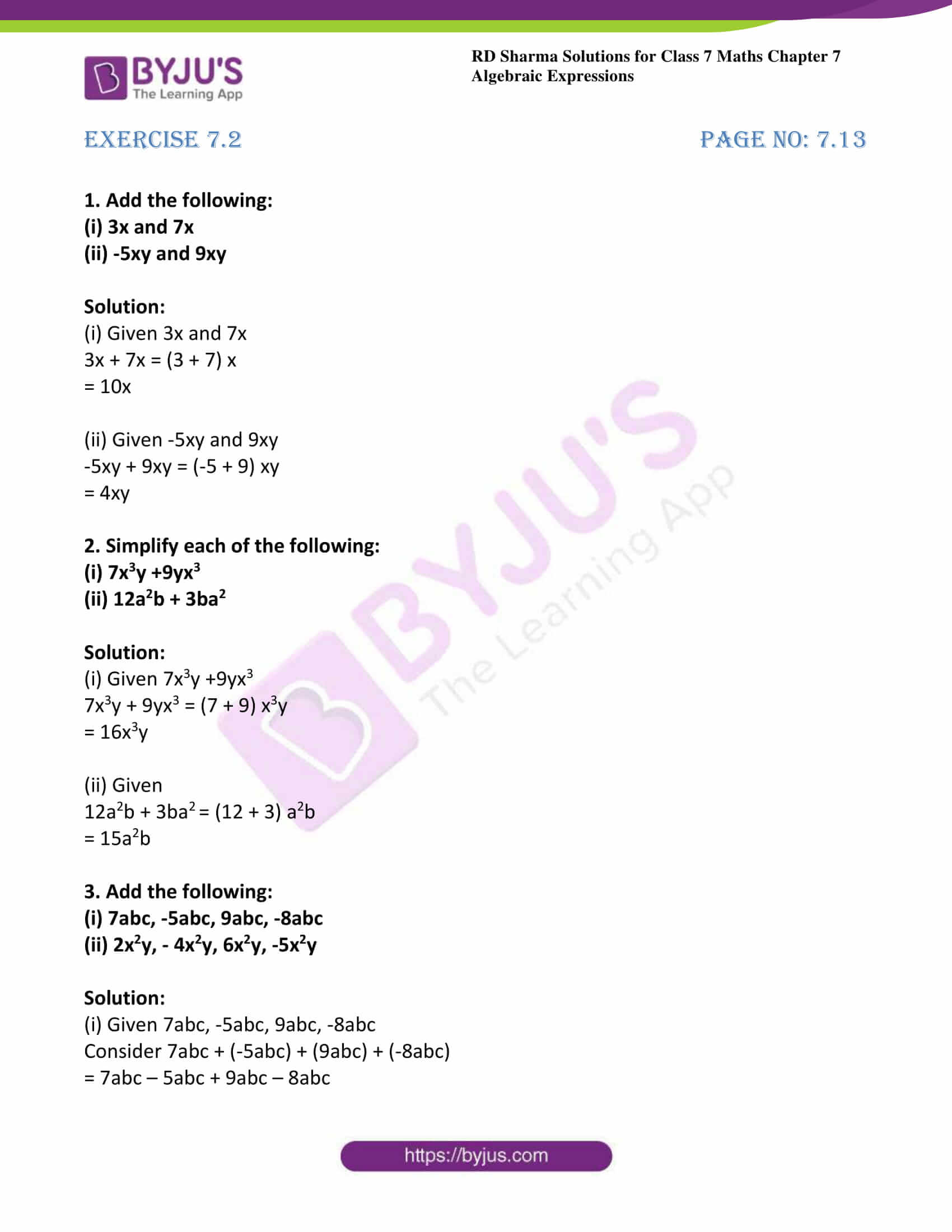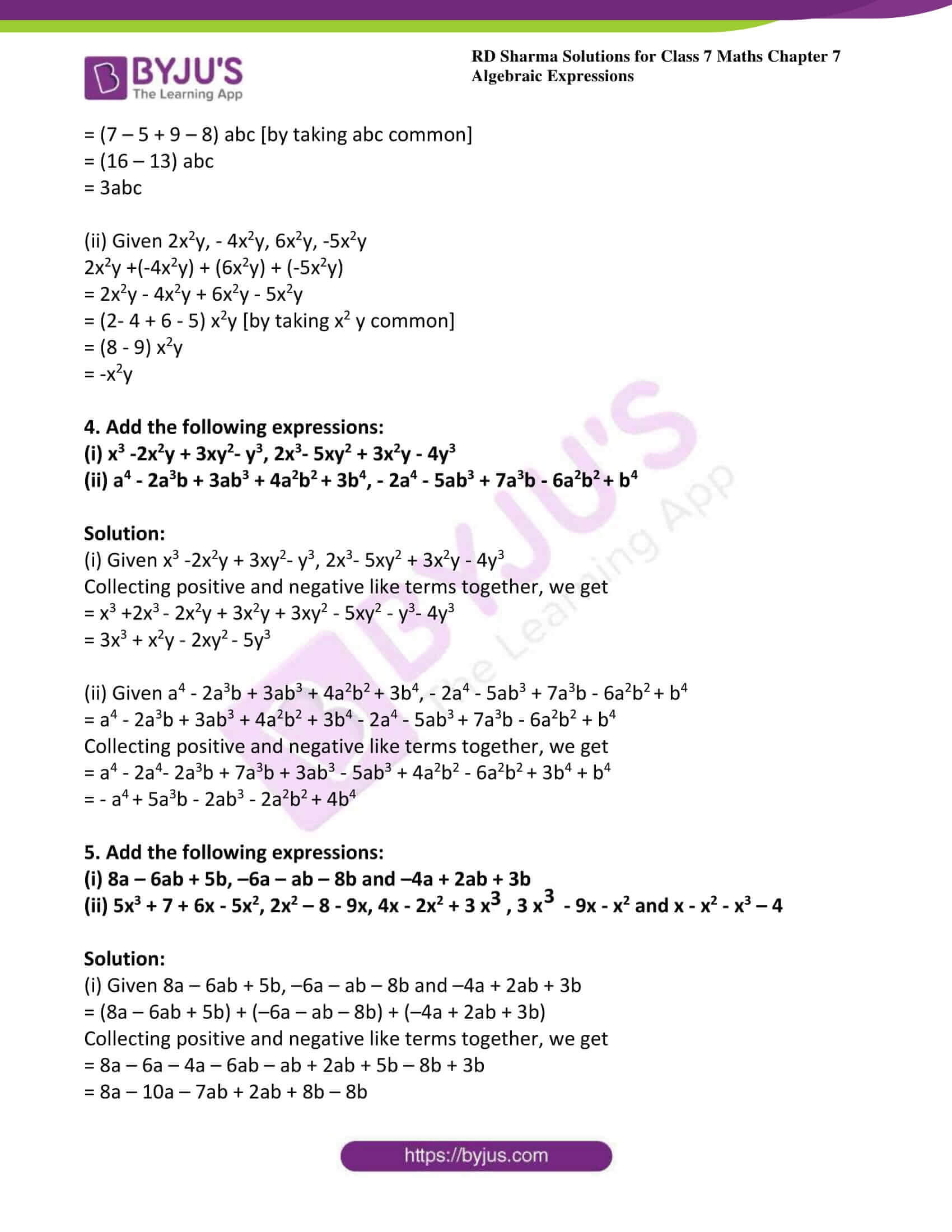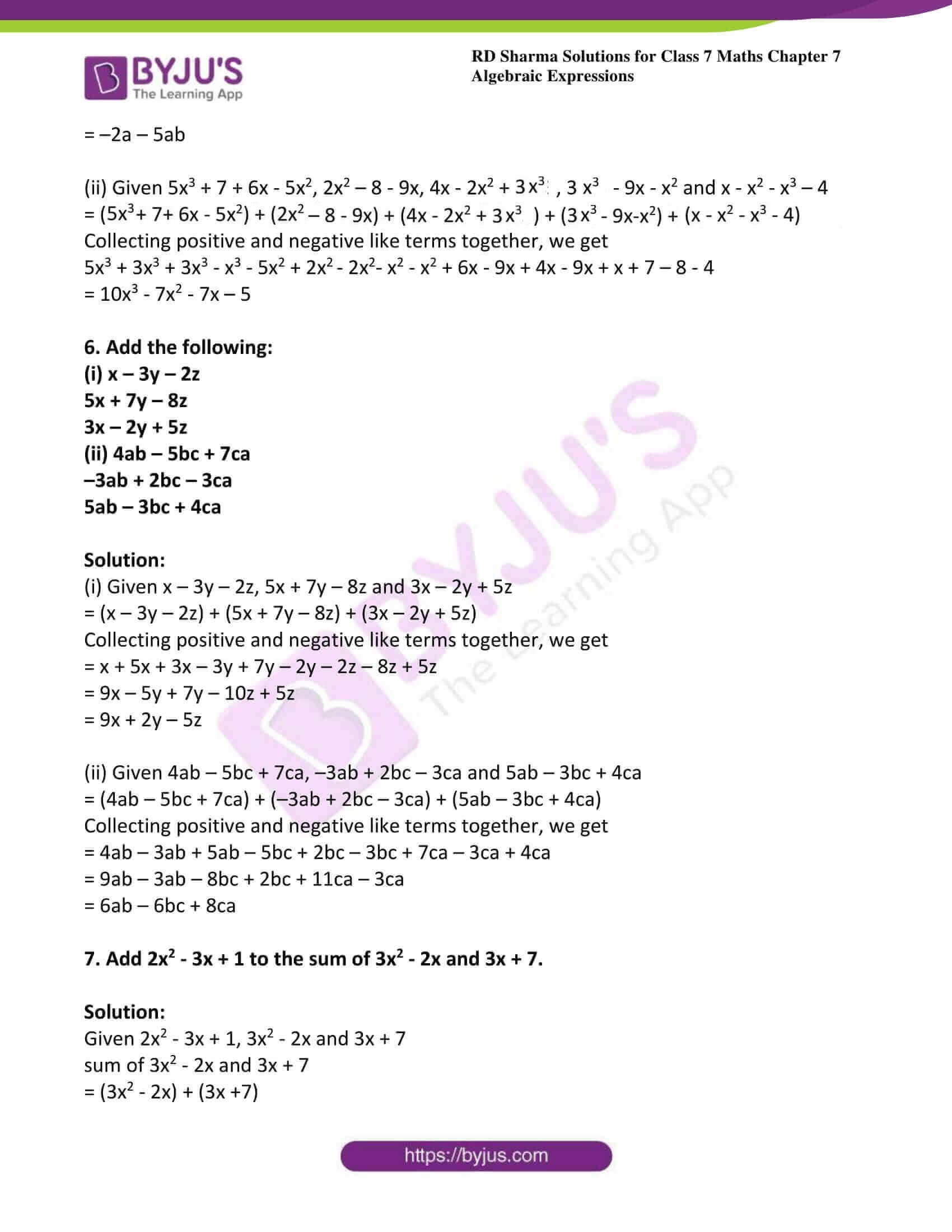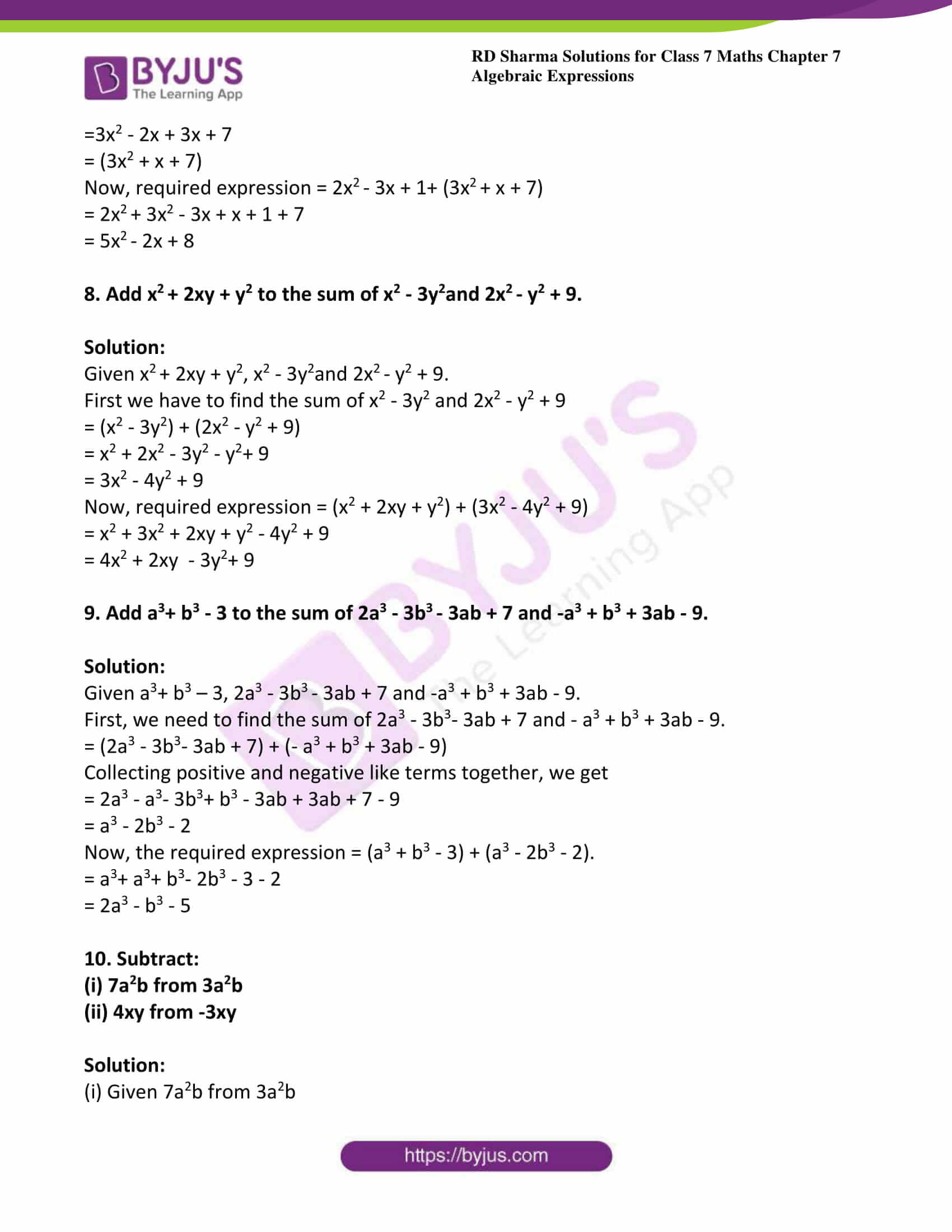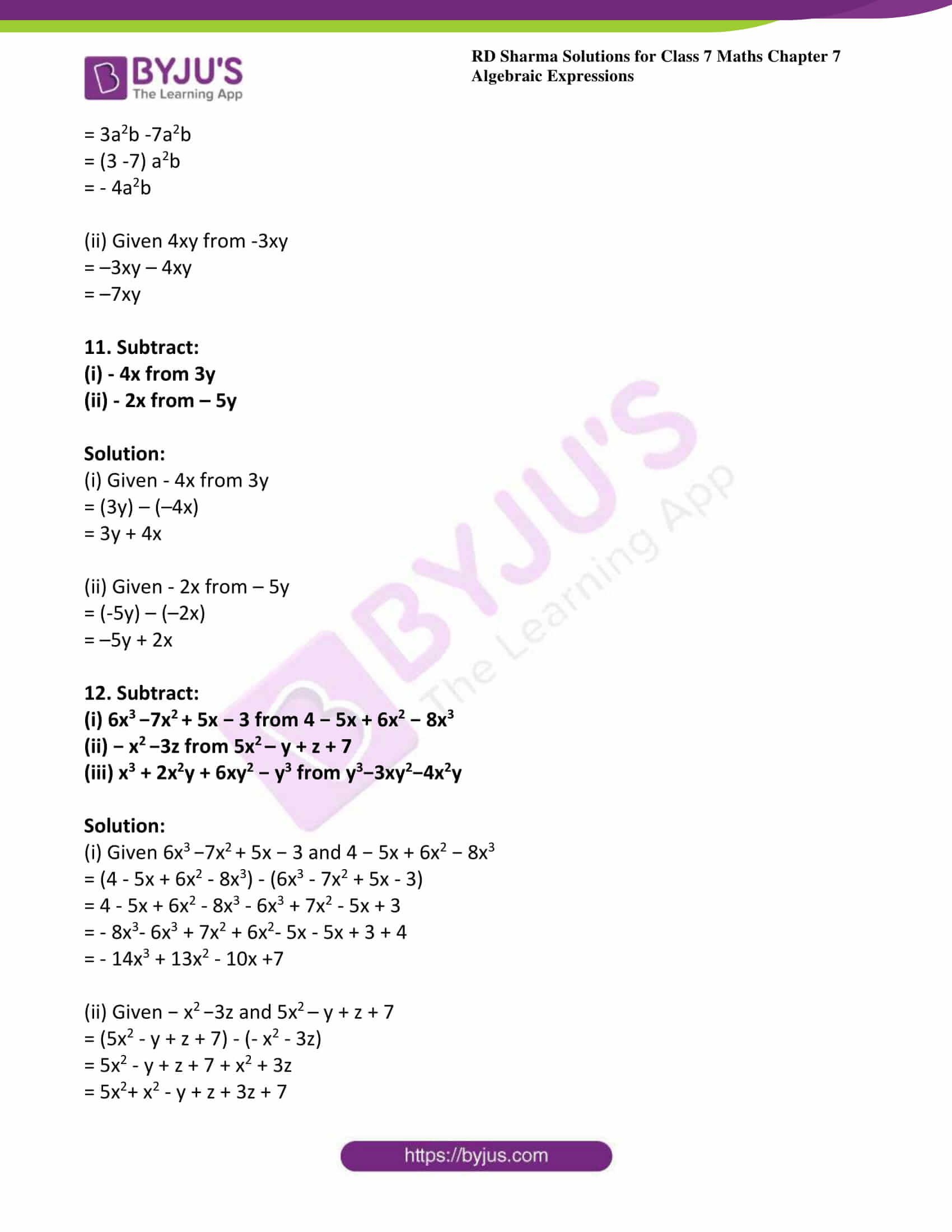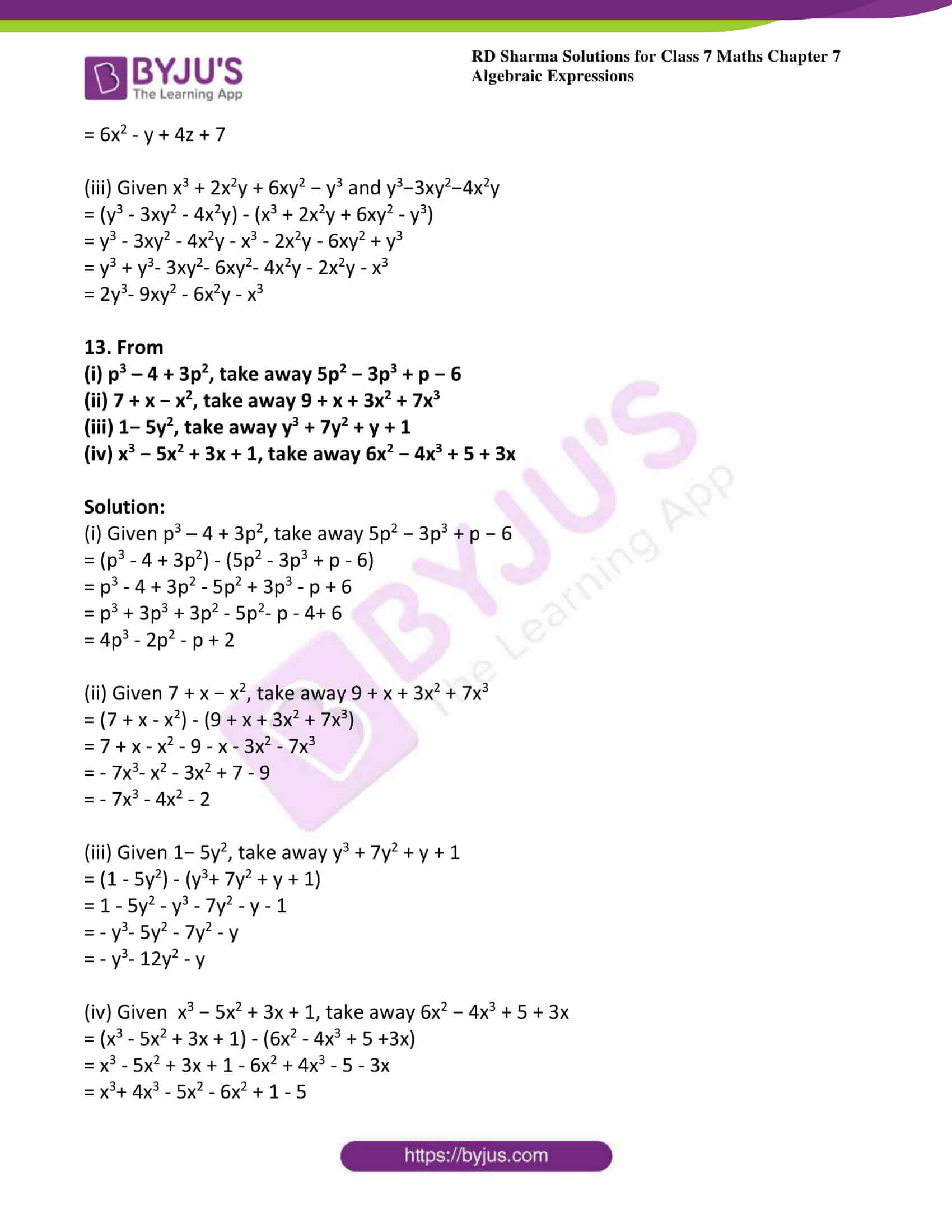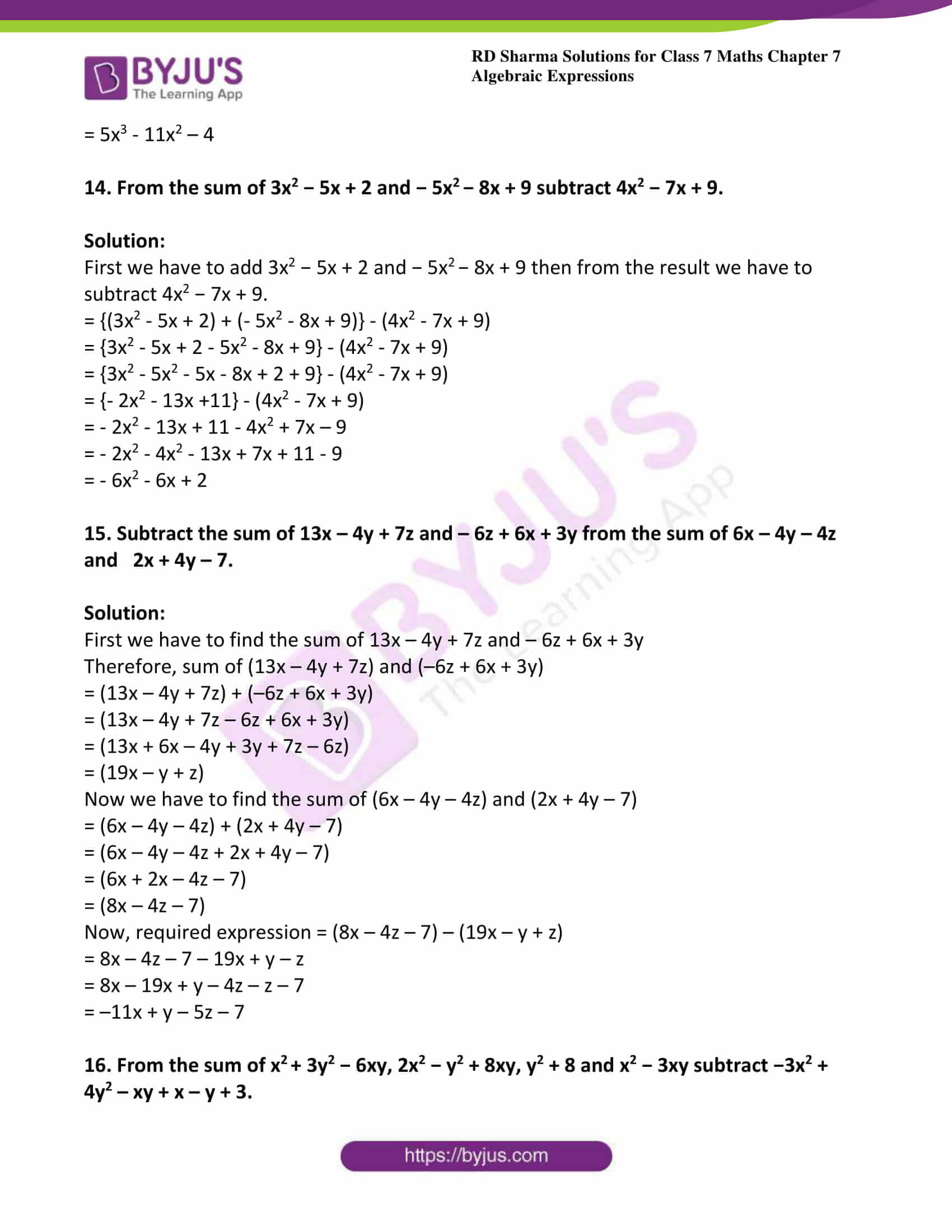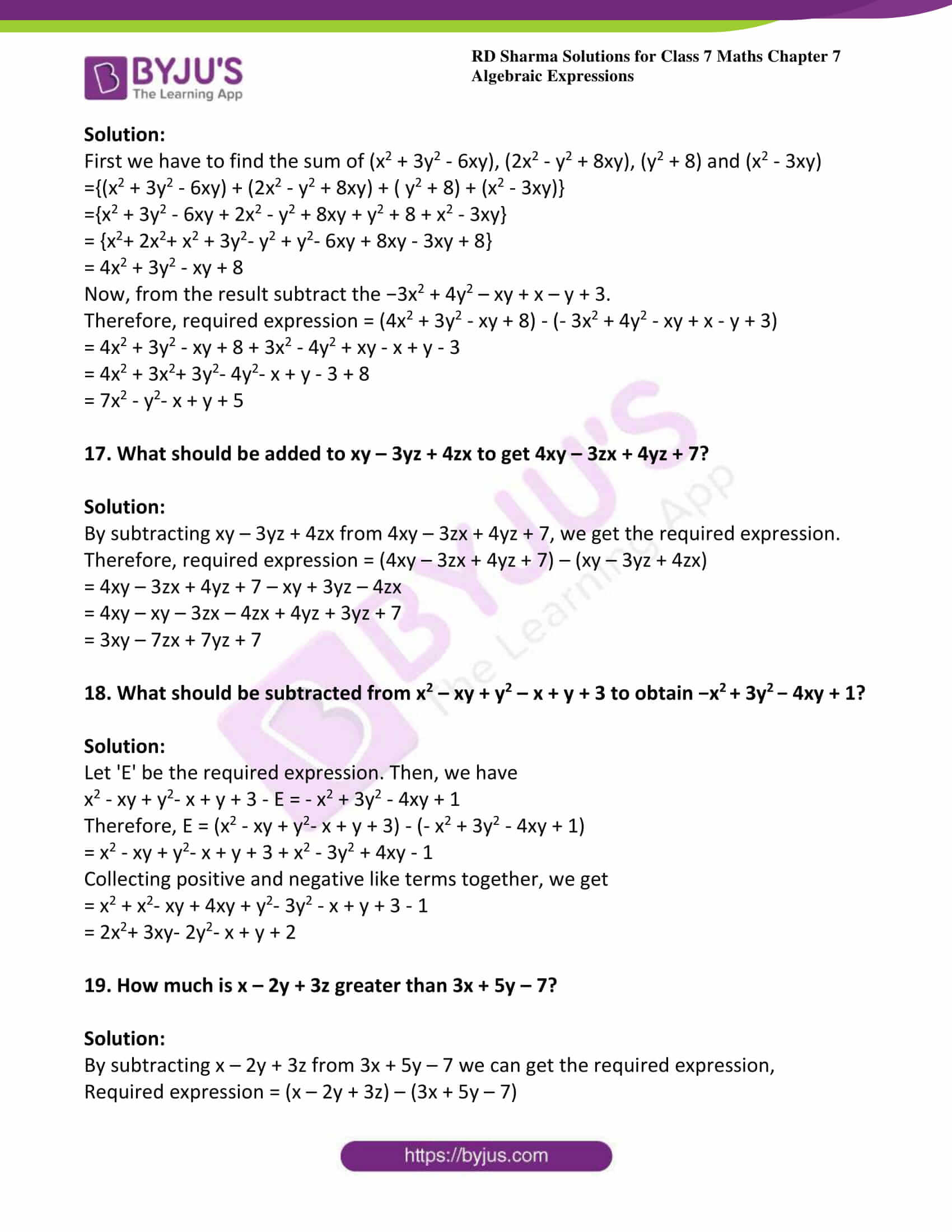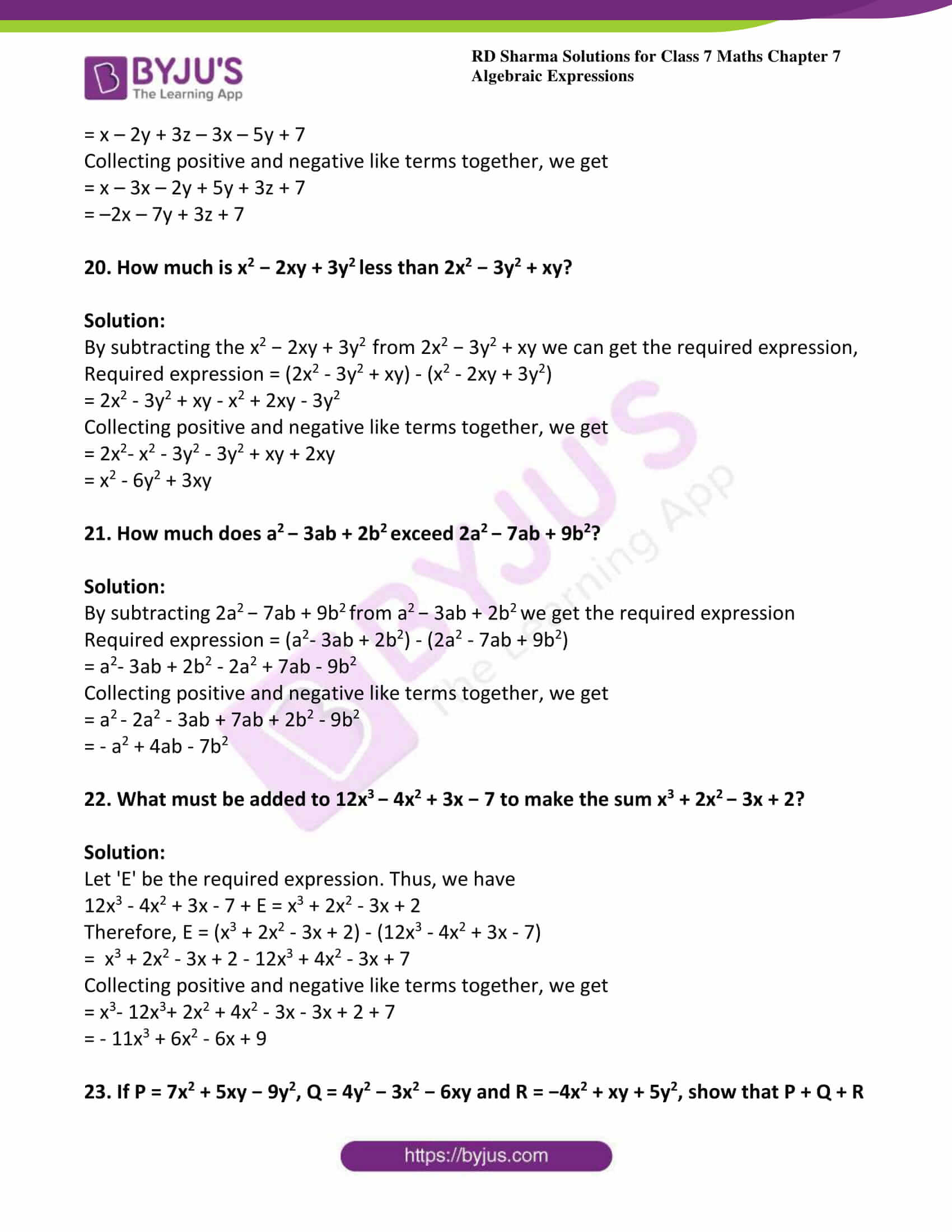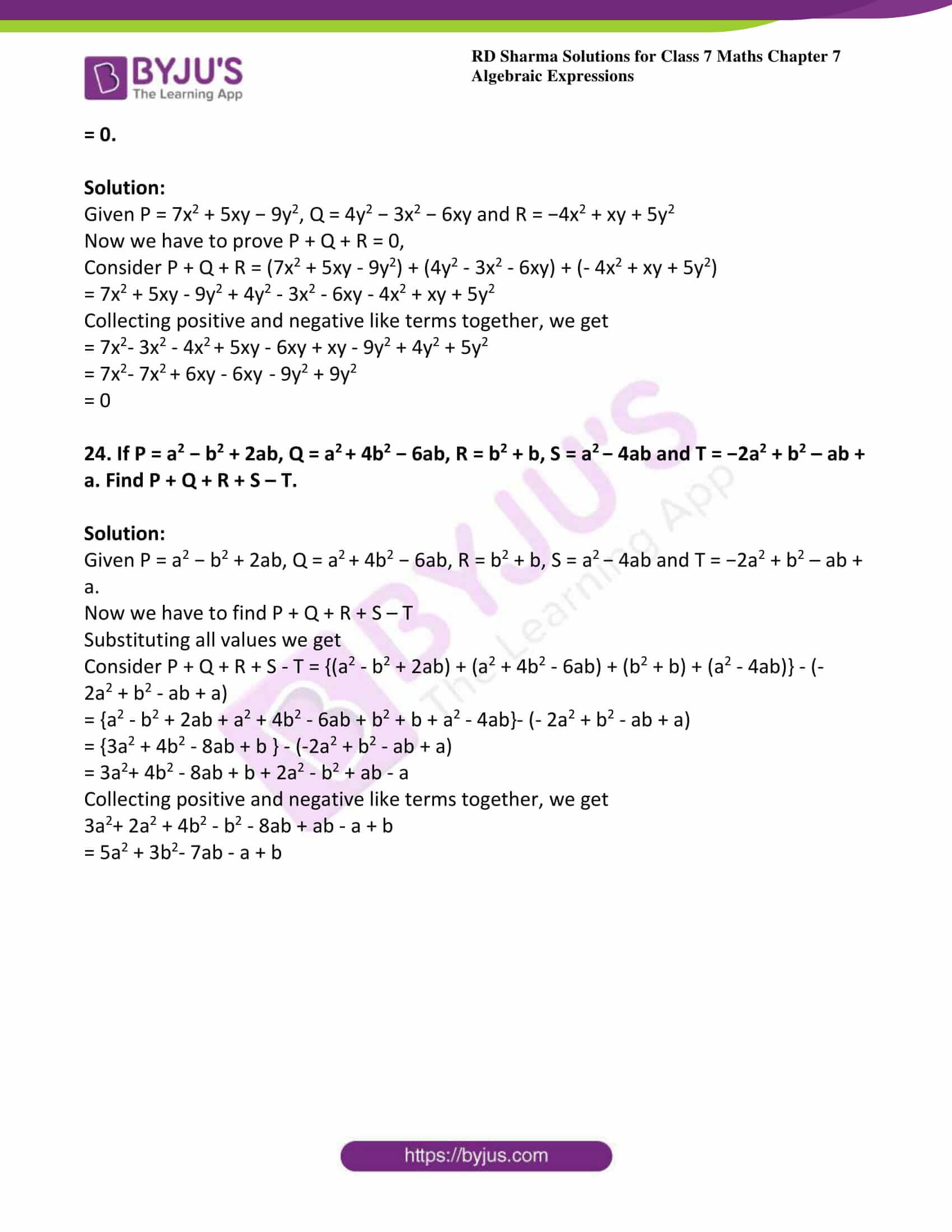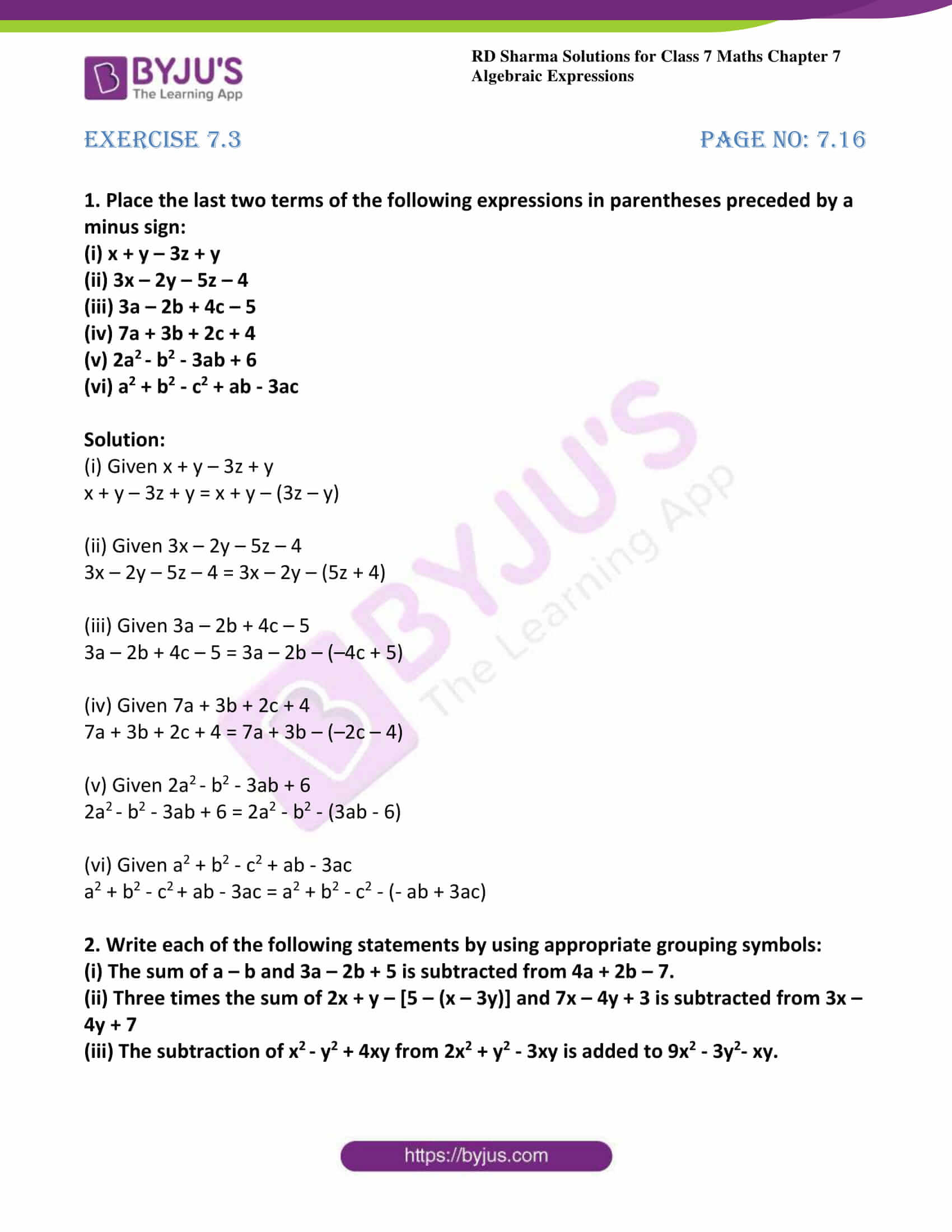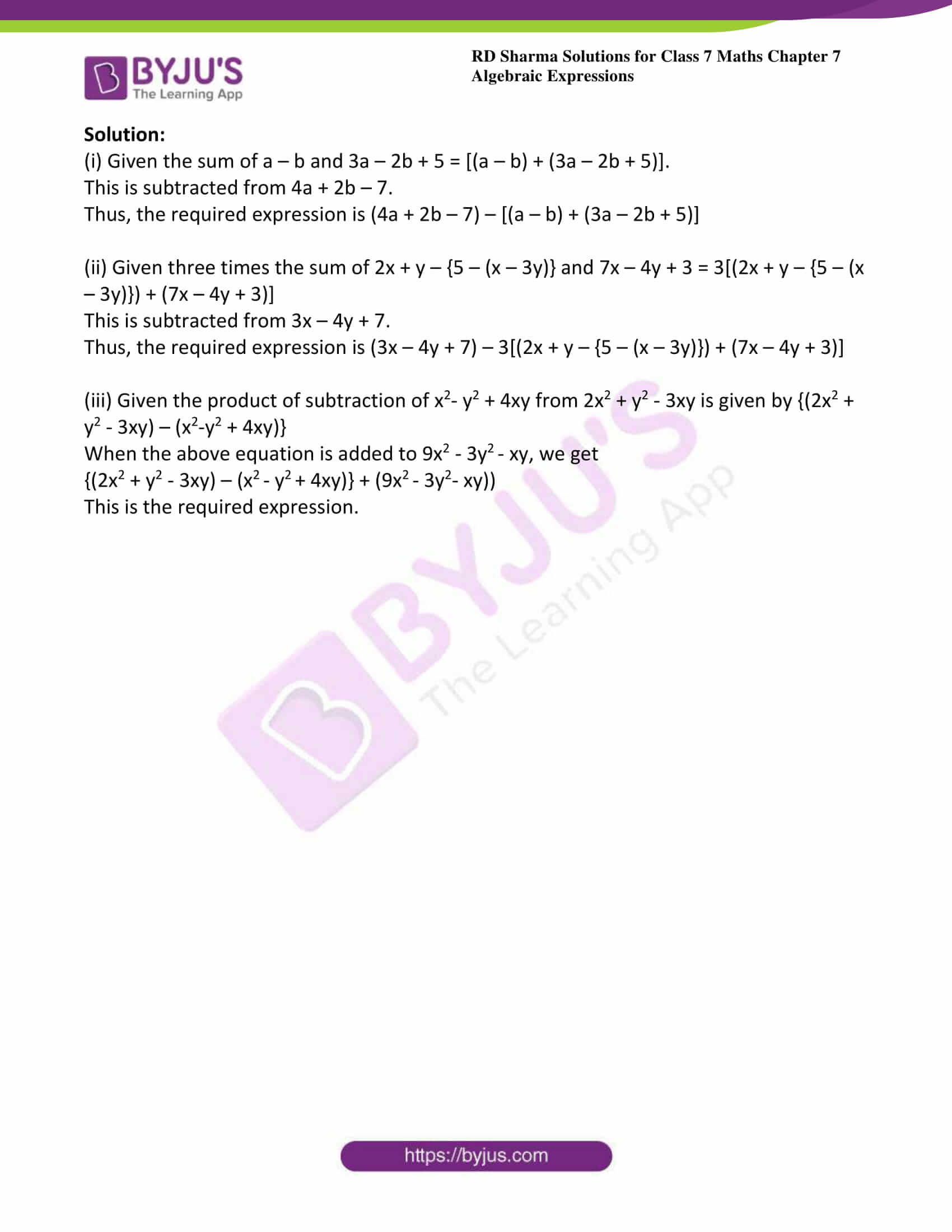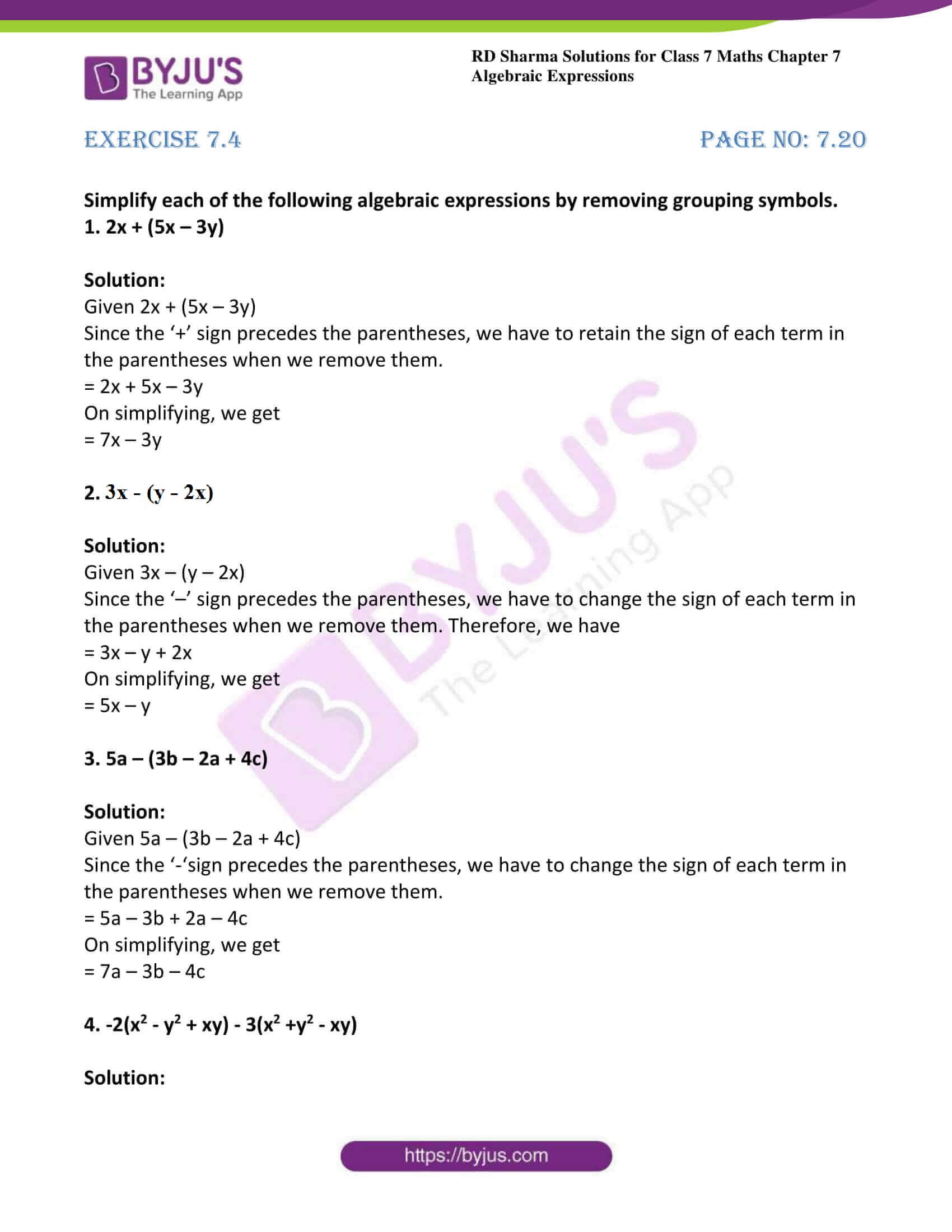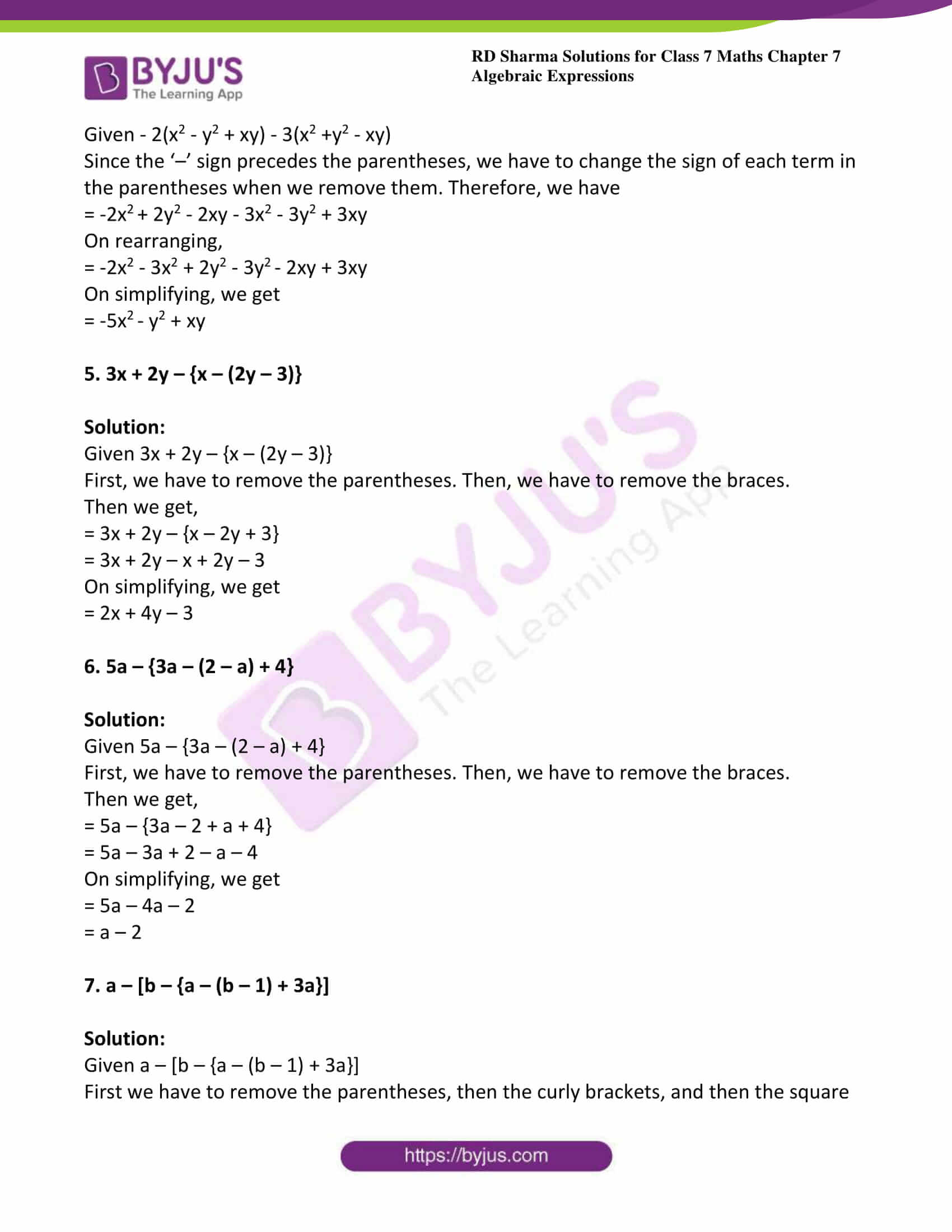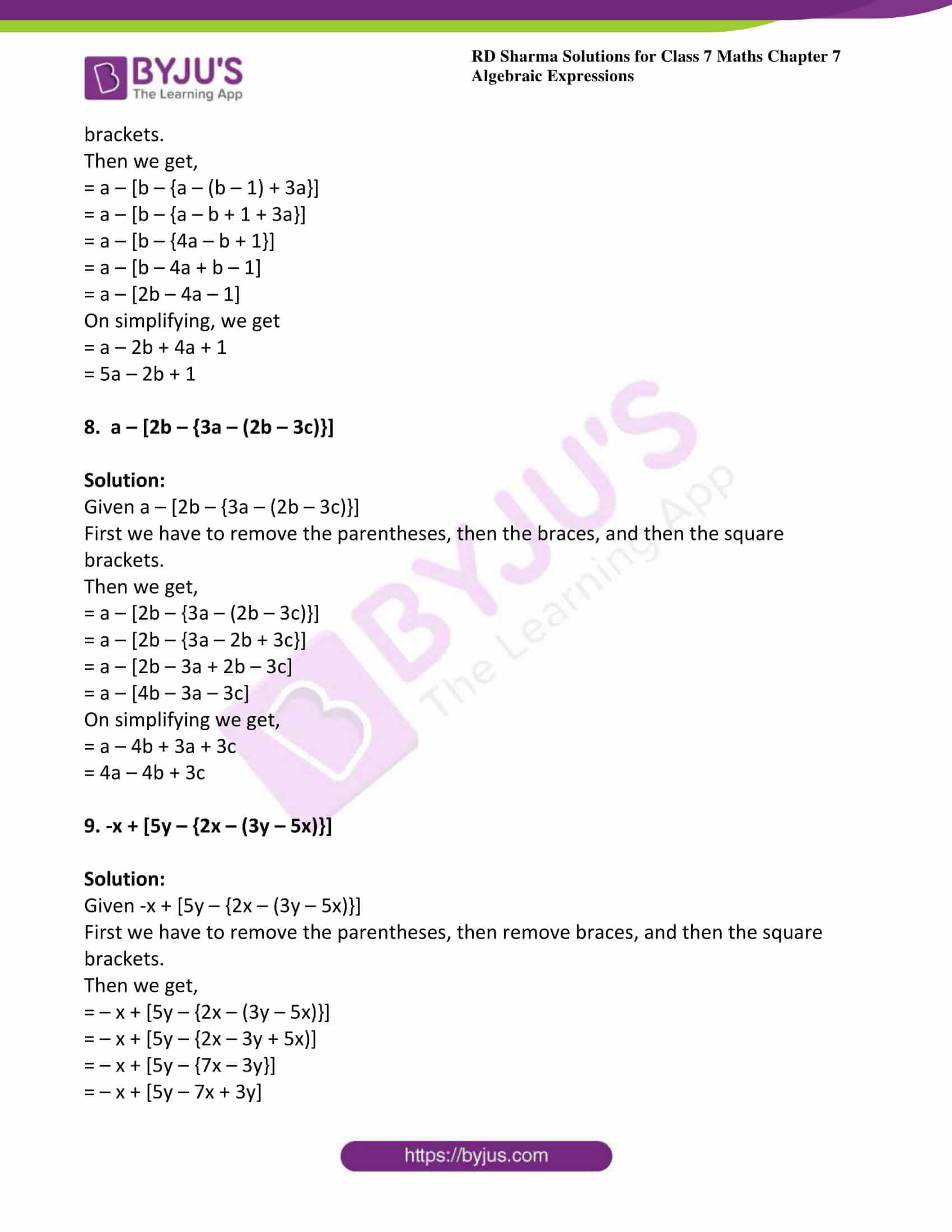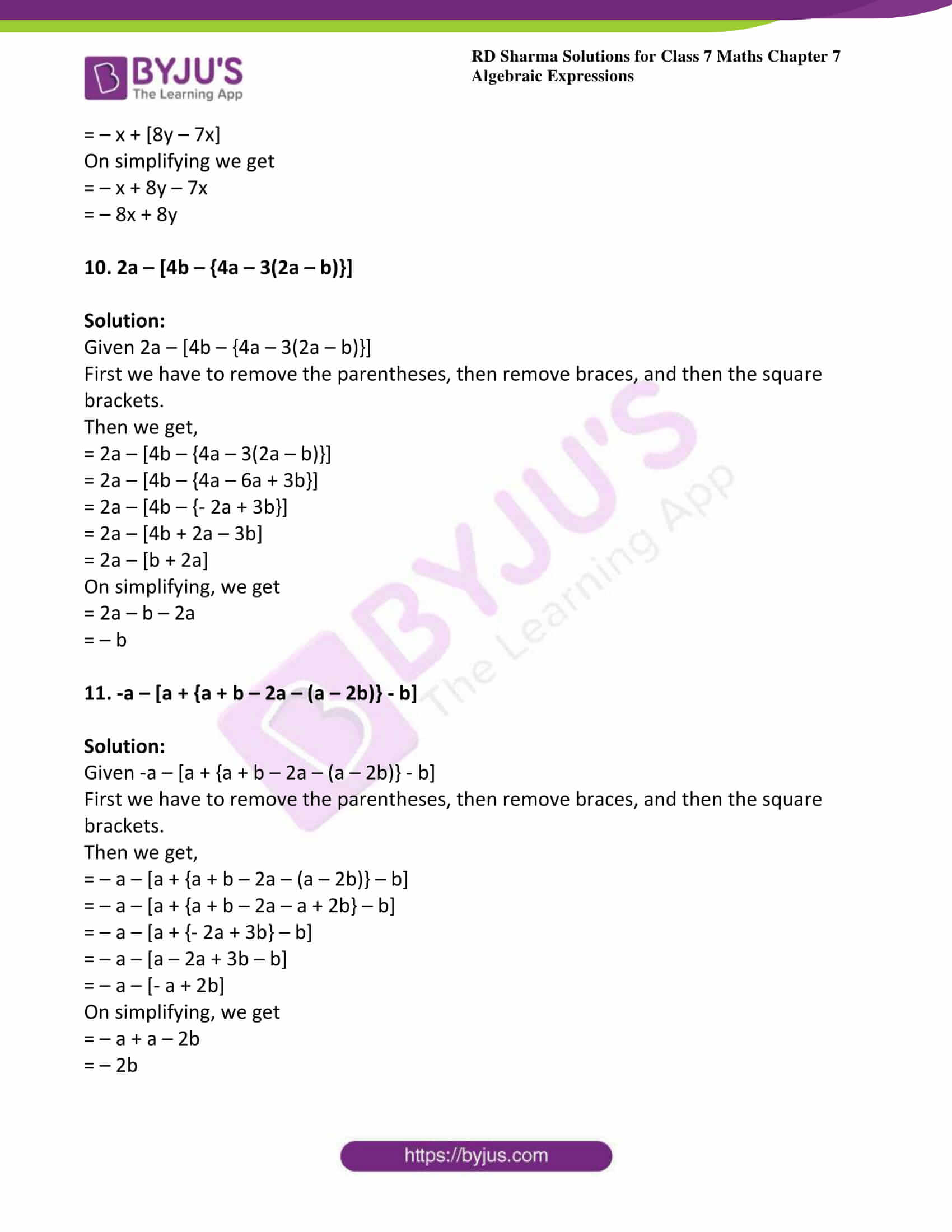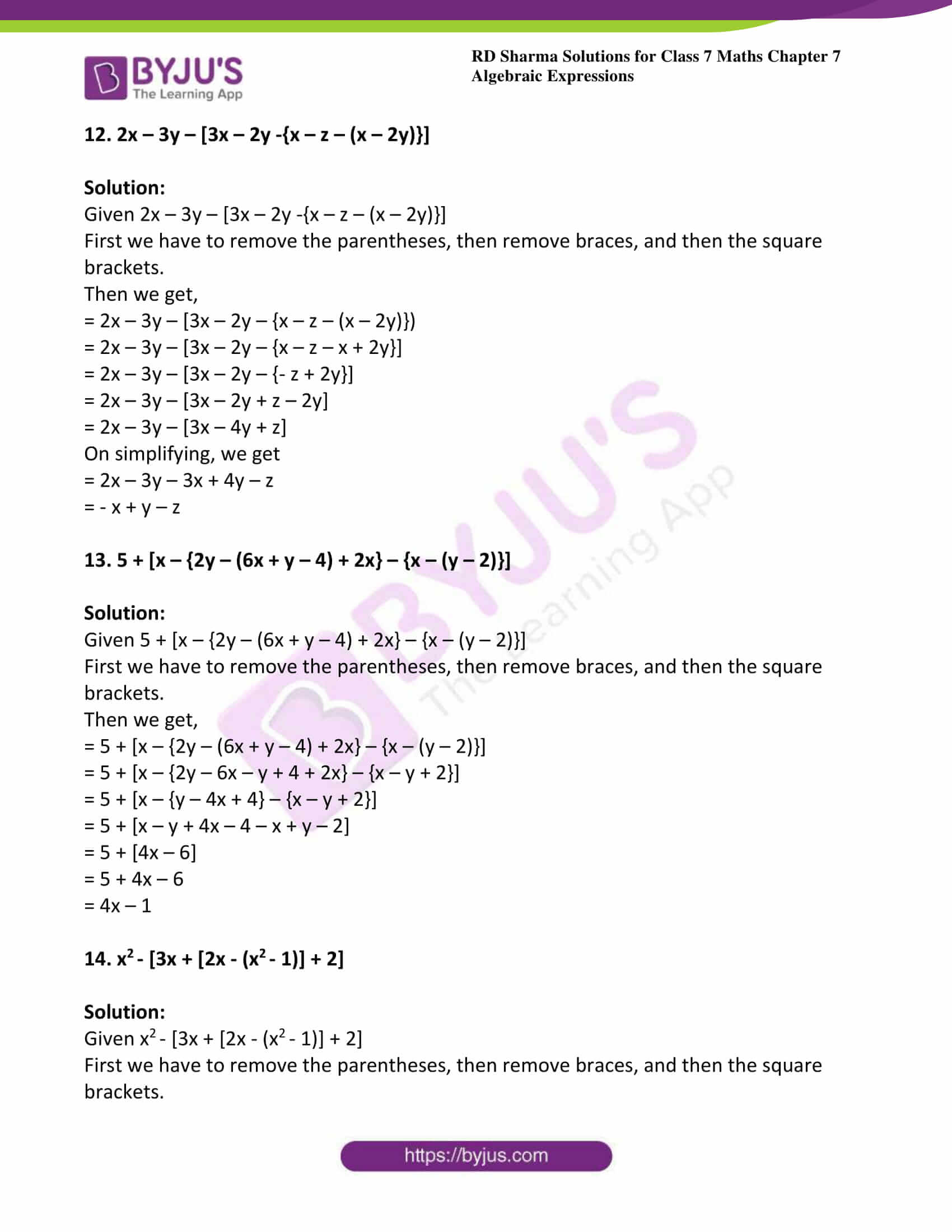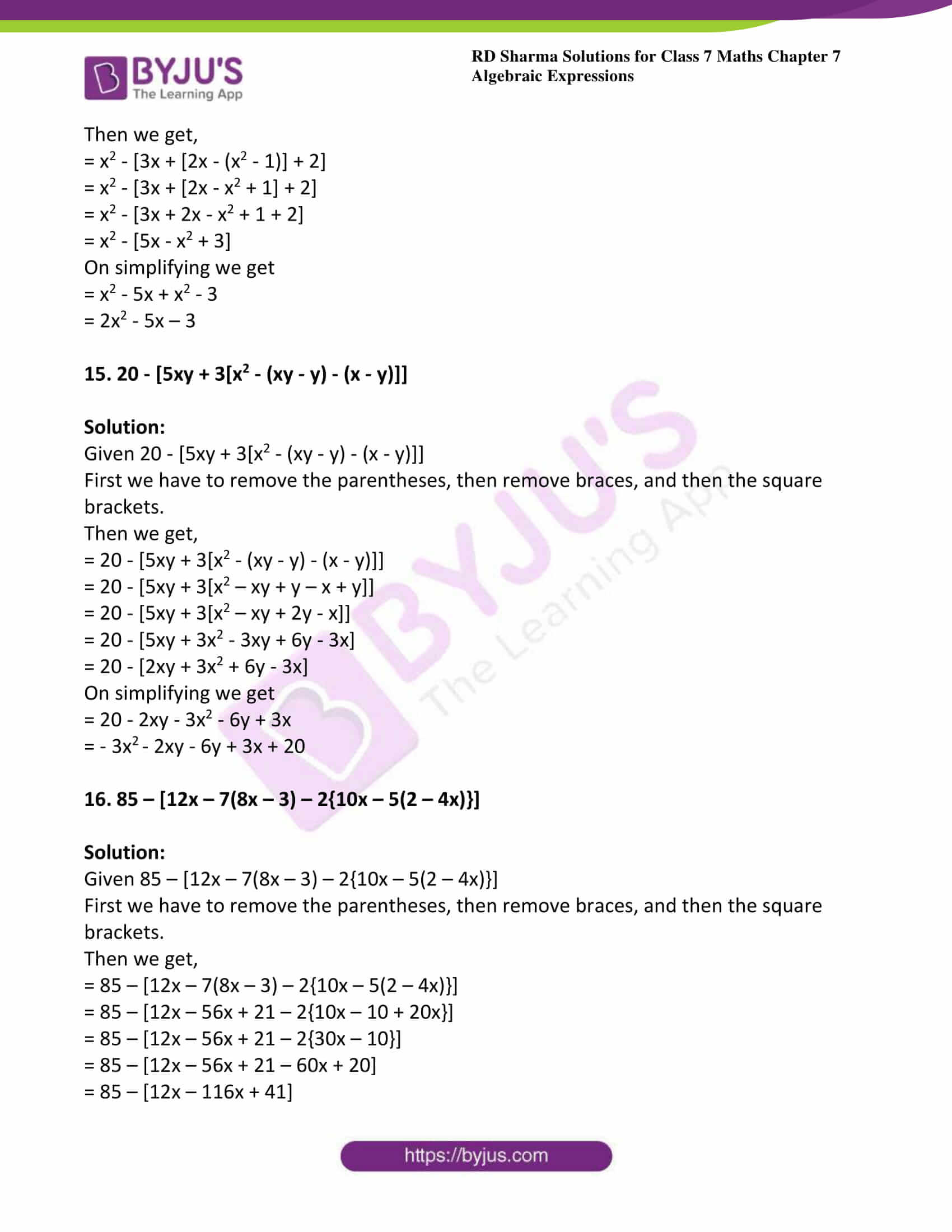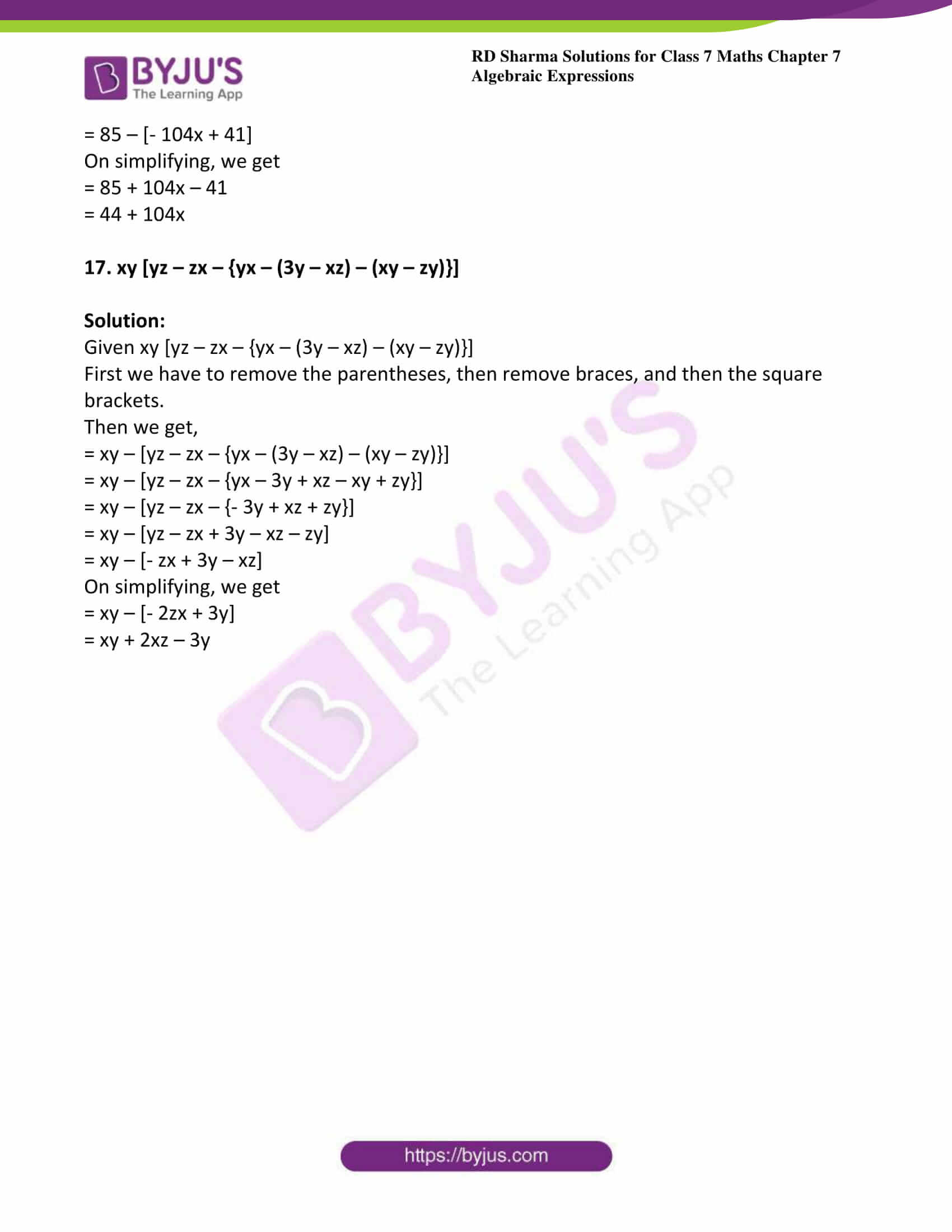### Access answers to Maths RD Sharma Solutions For Class 7 Chapter 7 – Algebraic Expressions

Exercise 7.1 Page No: 7.7

1. Identify the monomials, binomials, trinomials and quadrinomials from the following expressions:

(i) a2

(ii) a2 − b2

(iii) x3 + y3 + z3

(iv) x+ y+ z3 + 3xyz

(v) 7 + 5

(vi) a b c + 1

(vii) 3x – 2 + 5

(viii) 2x – 3y + 4

(ix) x y + y z + z x

(x) ax3 + bx2 + cx + d

Solution:

(i) Given a2

a2 is a monomial expression because it contains only one term

(ii) Given a2 − b2

a− b2 is a binomial expression because it contains two terms

(iii) Given x3 + y3 + z3

x3 + y3 + z3 is a trinomial because it contains three terms

(iv) Given x+ y+ z3 + 3xyz

x3 + y+ z3 + 3xyz is a quadrinomial expression because it contains four terms

(v) Given 7 + 5

7 + 5 is a monomial expression because it contains only one term

(vi) Given a b c + 1

a b c + 1 is a binomial expression because it contains two terms

(vii) Given 3x – 2 + 5

3x – 2 + 5 is a binomial expression because it contains two terms

(viii) Given 2x – 3y + 4

2x – 3y + 4 is a trinomial because it contains three terms

(ix) Given x y + y z + z x

x y + y z + z x is a trinomial because it contains three terms

(x) Given ax3 + bx2 + cx + d

ax3 + bx2 + cx + d is a quadrinomial expression because it contains four terms

2. Write all the terms of each of the following algebraic expressions:

(i) 3x

(ii) 2x – 3

(iii) 2x2 − 7

(iv) 2x+ y− 3xy + 4

Solution:

(i) Given 3x

3x is the only term of the given algebraic expression.

(ii) Given 2x – 3

2x and -3 are the terms of the given algebraic expression.

(iii) Given 2x2 − 7

2x2 and −7 are the terms of the given algebraic expression.

(iv) Given 2x+ y− 3xy + 4

2x2, y2, −3xy and 4 are the terms of the given algebraic expression.

3. Identify the terms and also mention the numerical coefficients of those terms:

(i) 4xy, -5x2y, -3yx, 2xy2

(ii) 7a2bc,-3ca2b,-(5/2) abc2, 3/2abc2,(-4/3)cba2

Solution:

(i) Like terms 4xy, -3yx and Numerical coefficients 4, -3

(ii) Like terms (7a2bc, −3ca2b) and (−4/3cba2) and their Numerical coefficients 7, -3,

(-4/3)

Like terms are (−5/2abc2) and (3/2 abc2) and numerical coefficients are (−5/2) and (3/2)

4. Identify the like terms in the following algebraic expressions:

(i) a2 + b-2a2 + c2 + 4a

(ii) 3x + 4xy − 2yz + 52zy

(iii) abc + ab2c + 2acb+ 3c2ab + b2ac − 2a2bc + 3cab2

Solution:

(i) Given a2 + b-2a2 + c2 + 4a

The like terms in the given algebraic expressions are a2 and −2a2.

(ii) Given 3x + 4xy − 2yz + 52zy

The like terms in the given algebraic expressions are -2yz and 52zy.

(iii) Given abc + ab2c + 2acb+ 3c2ab + b2ac − 2a2bc + 3cab2

The like terms in the given algebraic expressions are ab2c, 2acb2, b2ac and 3cab2.

5. Write the coefficient of x in the following:

(i) –12x

(ii) –7xy

(iii) xyz

(iv) –7ax

Solution:

(i) Given -12x

The numerical coefficient of x is -12.

(ii) Given -7xy

The numerical coefficient of x is -7y.

(iii) Given xyz

The numerical coefficient of x is yz.

(iv) Given -7ax

The numerical coefficient of x is -7a.

6. Write the coefficient of x2 in the following:

(i) −3x2

(ii) 5x2yz

(iii) 5/7x2z

(iv) (-3/2) ax2 + yx

Solution:

(i) Given −3x2

The numerical coefficient of x2 is -3.

(ii) Given 5x2yz

The numerical coefficient of x2 is 5yz.

(iii) Given 5/7x2z

The numerical coefficient of x2 is 5/7z.

(iv) Given (-3/2) ax2 + yx

The numerical coefficient of x2 is – (3/2) a.

7. Write the coefficient of:

(i) y in –3y

(ii) a in 2ab

(iii) z in –7xyz

(iv) p in –3pqr

(v) y2 in 9xy2z

(vi) x3 in x3 +1

(vii) x2 in − x2

Solution:

(i) Given –3y

The coefficient of y is -3.

(ii) Given 2ab

The coefficient of a is 2b.

(iii) Given -7xyz

The coefficient of z is -7xy.

(iv) Given -3pqr

The coefficient of p is -3qr.

(v) Given 9xy2z

The coefficient of y2 is 9xz.

(vi) Given x3 +1

The coefficient of x3 is 1.

(vii) Given − x2

The coefficient of x2 is -1.

8. Write the numerical coefficient of each in the following:

(i) xy

(ii) -6yz

(iii) 7abc

(iv) -2x3y2z

Solution:

(i) Given xy

The numerical coefficient in the term xy is 1.

(ii) Given -6yz

The numerical coefficient in the term – 6yz is – 6.

(iii) Given 7abc

The numerical coefficient in the term 7abc is 7.

(iv) Given -2x3y2z

The numerical coefficient in the term −2x3y2z is -2.

9. Write the numerical coefficient of each term in the following algebraic expressions:

(i) 4x2y – (3/2)xy + 5/2 xy2

(ii) (–5/3)x2y + (7/4)xyz + 3

Solution:

(i) Given 4x2y – (3/2) xy + 5/2 xy2

Numerical coefficient of following algebraic expressions are given below

 Term Coefficient 4x2y 4 – (3/2) xy -(3/2) 5/2 xy2 (5/2)

(ii) Given (–5/3)x2y + (7/4)xyz + 3

Numerical coefficient of following algebraic expressions are given below

 Term Coefficient (–5/3)x2y (-5/3) (7/4)xyz (7/4) 3 3

10. Write the constant term of each of the following algebraic expressions:

(i) x2y − xy2 + 7xy − 3

(ii) a3 − 3a2 + 7a + 5

Solution:

(i) Given x2y − xy2 + 7xy − 3

The constant term in the given algebraic expressions is -3.

(ii) Given a3 − 3a2 + 7a + 5

The constant term in the given algebraic expressions is 5.

11. Evaluate each of the following expressions for x = -2, y = -1, z = 3:

(i) (x/y) + (y/z) + (z/x)

(ii) x2 + y2 + z2 – xy – yz – zx

Solution:

(i) Given x = -2, y = -1, z = 3

Consider (x/y) + (y/z) + (z/x)

On substituting the given values we get,

= (-2/-1) + (-1/3) + (3/-2)

The LCM of 3 and 2 is 6

= (12 – 2 – 9)/6

= (1/6)

(ii) Given x = -2, y = -1, z = 3

Consider x2 + y2 + z2 – xy – yz – zx

On substituting the given values we get,

= (-2)2 + (-1)2 + 32 – (-2) (-1) – (-1) (3) – (3) (-2)

= 4 + 1 + 9 – 2 + 3 + 6

= 23 – 2

= 21

12. Evaluate each of the following algebraic expressions for x = 1, y = -1, z = 2, a = -2, b = 1, c = -2:

(i) ax + by + cz

(ii) ax2 + by2 – cz

(iii) axy + byz + cxy

Solution:

(i) Given x = 1, y = -1, z = 2, a = -2, b = 1, c = -2

Consider ax + by + cz

On substituting the given values

= (-2) (1) + (1) (-1) + (-2) (2)

= –2 – 1 – 4

= –7

(ii) Given x = 1, y = -1, z = 2, a = -2, b = 1, c = -2

Consider ax2 + by2 – cz

On substituting the given values

= (-2) × 12 + 1 × (-1)2 – (-2) × 2

= -2 + 1 – (-4)

= -1 + 4

= 3

(iii) Given x = 1, y = -1, z = 2, a = -2, b = 1, c = -2

Consider axy + byz + cxy

= (-2) × 1 × -1 + 1 × -1 × 2 + (-2) × 1 × (-1)

= 2 + (-2) + 2

= 4 – 2

= 2

Exercise 7.2 Page No: 7.13

(i) 3x and 7x

(ii) -5xy and 9xy

Solution:

(i) Given 3x and 7x

3x + 7x = (3 + 7) x

= 10x

(ii) Given -5xy and 9xy

-5xy + 9xy = (-5 + 9) xy

= 4xy

2. Simplify each of the following:

(i) 7x3y +9yx3

(ii) 12a2b + 3ba2

Solution:

(i) Given 7x3y +9yx3

7x3y + 9yx3 = (7 + 9) x3y

= 16x3y

(ii) Given

12a2b + 3ba= (12 + 3) a2b

= 15a2b

(i) 7abc, -5abc, 9abc, -8abc

(ii) 2x2y, – 4x2y, 6x2y, -5x2y

Solution:

(i) Given 7abc, -5abc, 9abc, -8abc

Consider 7abc + (-5abc) + (9abc) + (-8abc)

= 7abc – 5abc + 9abc – 8abc

= (7 – 5 + 9 – 8) abc [by taking abc common]

= (16 – 13) abc

= 3abc

(ii) Given 2x2y, – 4x2y, 6x2y, -5x2y

2x2y +(-4x2y) + (6x2y) + (-5x2y)

= 2x2y – 4x2y + 6x2y – 5x2y

= (2- 4 + 6 – 5) x2y [by taking x2 y common]

= (8 – 9) x2y

= -x2y

(i) x3 -2x2y + 3xy2– y3, 2x3– 5xy2 + 3x2y – 4y3

(ii) a4 – 2a3b + 3ab3 + 4a2b+ 3b4, – 2a4 – 5ab3 + 7a3b – 6a2b+ b4

Solution:

(i) Given x3 -2x2y + 3xy2– y3, 2x3– 5xy2 + 3x2y – 4y3

Collecting positive and negative like terms together, we get

= x3 +2x– 2x2y + 3x2y + 3xy2 – 5xy2 – y3– 4y3

= 3x3 + x2y – 2xy– 5y3

(ii) Given a4 – 2a3b + 3ab3 + 4a2b+ 3b4, – 2a4 – 5ab3 + 7a3b – 6a2b+ b4

= a4 – 2a3b + 3ab3 + 4a2b2 + 3b4 – 2a4 – 5ab+ 7a3b – 6a2b2 + b4

Collecting positive and negative like terms together, we get

= a4 – 2a4– 2a3b + 7a3b + 3ab3 – 5ab3 + 4a2b2 – 6a2b+ 3b4 + b4

= – a+ 5a3b – 2ab3 – 2a2b+ 4b4

(i) 8a – 6ab + 5b, –6a – ab – 8b and –4a + 2ab + 3b

(ii) 5x3 + 7 + 6x – 5x2, 2x2 – 8 – 9x, 4x – 2x2 + 3 x 3, 3 x 3 – 9x – x2 and x – x2 – x3 – 4

Solution:

(i) Given 8a – 6ab + 5b, –6a – ab – 8b and –4a + 2ab + 3b

= (8a – 6ab + 5b) + (–6a – ab – 8b) + (–4a + 2ab + 3b)

Collecting positive and negative like terms together, we get

= 8a – 6a – 4a – 6ab – ab + 2ab + 5b – 8b + 3b

= 8a – 10a – 7ab + 2ab + 8b – 8b

= –2a – 5ab

(ii) Given 5x3 + 7 + 6x – 5x2, 2x2 – 8 – 9x, 4x – 2x2 + 3 x 3, 3 x 3 – 9x – x2 and x – x2 – x3 – 4

= (5 x 3 + 7+ 6x – 5x2) + (2 x 2 – 8 – 9x) + (4x – 2x2 + 3 x 3) + (3 x 3 – 9x-x2) + (x – x2 – x3 – 4)

Collecting positive and negative like terms together, we get

5x3 + 3x3 + 3x3 – x3 – 5x2 + 2x– 2x2– x2 – x2 + 6x – 9x + 4x – 9x + x + 7 – 8 – 4

= 10x3 – 7x2 – 7x – 5

(i) x – 3y – 2z

5x + 7y – 8z

3x – 2y + 5z

(ii) 4ab – 5bc + 7ca

–3ab + 2bc – 3ca

5ab – 3bc + 4ca

Solution:

(i) Given x – 3y – 2z, 5x + 7y – 8z and 3x – 2y + 5z

= (x – 3y – 2z) + (5x + 7y – 8z) + (3x – 2y + 5z)

Collecting positive and negative like terms together, we get

= x + 5x + 3x – 3y + 7y – 2y – 2z – 8z + 5z

= 9x – 5y + 7y – 10z + 5z

= 9x + 2y – 5z

(ii) Given 4ab – 5bc + 7ca, –3ab + 2bc – 3ca and 5ab – 3bc + 4ca

= (4ab – 5bc + 7ca) + (–3ab + 2bc – 3ca) + (5ab – 3bc + 4ca)

Collecting positive and negative like terms together, we get

= 4ab – 3ab + 5ab – 5bc + 2bc – 3bc + 7ca – 3ca + 4ca

= 9ab – 3ab – 8bc + 2bc + 11ca – 3ca

= 6ab – 6bc + 8ca

7. Add 2x2 – 3x + 1 to the sum of 3x2 – 2x and 3x + 7.

Solution:

Given 2x2 – 3x + 1, 3x2 – 2x and 3x + 7

sum of 3x2 – 2x and 3x + 7

= (3x2 – 2x) + (3x +7)

=3x2 – 2x + 3x + 7

= (3x2 + x + 7)

Now, required expression = 2x– 3x + 1+ (3x+ x + 7)

= 2x+ 3x2 – 3x + x + 1 + 7

= 5x– 2x + 8

8. Add x+ 2xy + y2 to the sum of x2 – 3y2and 2x– y2 + 9.

Solution:

Given x+ 2xy + y2, x2 – 3y2and 2x– y2 + 9.

First we have to find the sum of x2 – 3y2 and 2x2 – y2 + 9

= (x2 – 3y2) + (2x2 – y2 + 9)

= x2 + 2x2 – 3y2 – y2+ 9

= 3x2 – 4y2 + 9

Now, required expression = (x2 + 2xy + y2) + (3x2 – 4y2 + 9)

= x2 + 3x2 + 2xy + y2 – 4y2 + 9

= 4x2 + 2xy  – 3y2+ 9

9. Add a3+ b3 – 3 to the sum of 2a3 – 3b– 3ab + 7 and -a3 + b3 + 3ab – 9.

Solution:

Given a3+ b3 – 3, 2a3 – 3b– 3ab + 7 and -a3 + b3 + 3ab – 9.

First, we need to find the sum of 2a3 – 3b3– 3ab + 7 and – a3 + b3 + 3ab – 9.

= (2a3 – 3b3– 3ab + 7) + (- a3 + b3 + 3ab – 9)

Collecting positive and negative like terms together, we get

= 2a3 – a3– 3b3+ b3 – 3ab + 3ab + 7 – 9

= a3 – 2b3 – 2

Now, the required expression = (a3 + b3 – 3) + (a3 – 2b3 – 2).

= a3+ a3+ b3– 2b3 – 3 – 2

= 2a3 – b3 – 5

10. Subtract:

(i) 7a2b from 3a2b

(ii) 4xy from -3xy

Solution:

(i) Given 7a2b from 3a2b

= 3a2b -7a2b

= (3 -7) a2b

= – 4a2b

(ii) Given 4xy from -3xy

= –3xy – 4xy

= –7xy

11. Subtract:

(i) – 4x from 3y

(ii) – 2x from – 5y

Solution:

(i) Given – 4x from 3y

= (3y) – (–4x)

= 3y + 4x

(ii) Given – 2x from – 5y

= (-5y) – (–2x)

= –5y + 2x

12. Subtract:

(i) 6x−7x+ 5x − 3 from 4 − 5x + 6x2 − 8x3

(ii) − x−3z from 5x– y + z + 7

(iii) x3 + 2x2y + 6xy2 − y3 from y3−3xy2−4x2y

Solution:

(i) Given 6x−7x+ 5x − 3 and 4 − 5x + 6x2 − 8x3

= (4 – 5x + 6x2 – 8x3) – (6x3 – 7x2 + 5x – 3)

= 4 – 5x + 6x2 – 8x3 – 6x3 + 7x2 – 5x + 3

= – 8x3– 6x3 + 7x2 + 6x2– 5x – 5x + 3 + 4

= – 14x3 + 13x2 – 10x +7

(ii) Given − x−3z and 5x– y + z + 7

= (5x2 – y + z + 7) – (- x2 – 3z)

= 5x2 – y + z + 7 + x2 + 3z

= 5x2+ x2 – y + z + 3z + 7

= 6x2 – y + 4z + 7

(iii) Given x3 + 2x2y + 6xy2 − y3 and y3−3xy2−4x2y

= (y3 – 3xy2 – 4x2y) – (x3 + 2x2y + 6xy2 – y3)

= y3 – 3xy2 – 4x2y – x3 – 2x2y – 6xy2 + y3

= y3 + y3– 3xy2– 6xy2– 4x2y – 2x2y – x3

= 2y3– 9xy2 – 6x2y – x3

13. From

(i) p3 – 4 + 3p2, take away 5p2 − 3p3 + p − 6

(ii) 7 + x − x2, take away 9 + x + 3x2 + 7x3

(iii) 1− 5y2, take away y3 + 7y2 + y + 1

(iv) x3 − 5x2 + 3x + 1, take away 6x2 − 4x3 + 5 + 3x

Solution:

(i) Given p3 – 4 + 3p2, take away 5p2 − 3p3 + p − 6

= (p3 – 4 + 3p2) – (5p2 – 3p3 + p – 6)

= p3 – 4 + 3p2 – 5p2 + 3p3 – p + 6

= p3 + 3p3 + 3p2 – 5p2– p – 4+ 6

= 4p3 – 2p2 – p + 2

(ii) Given 7 + x − x2, take away 9 + x + 3x2 + 7x3

= (7 + x – x2) – (9 + x + 3x2 + 7x3)

= 7 + x – x2 – 9 – x – 3x2 – 7x3

= – 7x3– x2 – 3x2 + 7 – 9

= – 7x3 – 4x2 – 2

(iii) Given 1− 5y2, take away y3 + 7y2 + y + 1

= (1 – 5y2) – (y3+ 7y2 + y + 1)

= 1 – 5y2 – y3 – 7y2 – y – 1

= – y3– 5y2 – 7y2 – y

= – y3– 12y2 – y

(iv) Given x3 − 5x2 + 3x + 1, take away 6x2 − 4x3 + 5 + 3x

= (x3 – 5x2 + 3x + 1) – (6x2 – 4x3 + 5 +3x)

= x3 – 5x2 + 3x + 1 – 6x2 + 4x3 – 5 – 3x

= x3+ 4x3 – 5x2 – 6x2 + 1 – 5

= 5x3 – 11x2 – 4

14. From the sum of 3x2 − 5x + 2 and − 5x− 8x + 9 subtract 4x2 − 7x + 9.

Solution:

First we have to add 3x2 − 5x + 2 and − 5x− 8x + 9 then from the result we have to subtract 4x2 − 7x + 9.

= {(3x2 – 5x + 2) + (- 5x2 – 8x + 9)} – (4x2 – 7x + 9)

= {3x2 – 5x + 2 – 5x2 – 8x + 9} – (4x2 – 7x + 9)

= {3x2 – 5x2 – 5x – 8x + 2 + 9} – (4x2 – 7x + 9)

= {- 2x2 – 13x +11} – (4x2 – 7x + 9)

= – 2x2 – 13x + 11 – 4x2 + 7x – 9

= – 2x2 – 4x2 – 13x + 7x + 11 – 9

= – 6x2 – 6x + 2

15. Subtract the sum of 13x – 4y + 7z and – 6z + 6x + 3y from the sum of 6x – 4y – 4z and   2x + 4y – 7.

Solution:

First we have to find the sum of 13x – 4y + 7z and – 6z + 6x + 3y

Therefore, sum of (13x – 4y + 7z) and (–6z + 6x + 3y)

= (13x – 4y + 7z) + (–6z + 6x + 3y)

= (13x – 4y + 7z – 6z + 6x + 3y)

= (13x + 6x – 4y + 3y + 7z – 6z)

= (19x – y + z)

Now we have to find the sum of (6x – 4y – 4z) and (2x + 4y – 7)

= (6x – 4y – 4z) + (2x + 4y – 7)

= (6x – 4y – 4z + 2x + 4y – 7)

= (6x + 2x – 4z – 7)

= (8x – 4z – 7)

Now, required expression = (8x – 4z – 7) – (19x – y + z)

= 8x – 4z – 7 – 19x + y – z

= 8x – 19x + y – 4z – z – 7

= –11x + y – 5z – 7

16. From the sum of x+ 3y2 − 6xy, 2x2 − y2 + 8xy, y2 + 8 and x2 − 3xy subtract −3x2 + 4y2 – xy + x – y + 3.

Solution:

First we have to find the sum of (x2 + 3y2 – 6xy), (2x2 – y2 + 8xy), (y2 + 8) and (x2 – 3xy)

={(x2 + 3y2 – 6xy) + (2x2 – y2 + 8xy) + ( y2 + 8) + (x2 – 3xy)}

={x2 + 3y2 – 6xy + 2x2 – y2 + 8xy + y2 + 8 + x2 – 3xy}

= {x2+ 2x2+ x2 + 3y2– y2 + y2– 6xy + 8xy – 3xy + 8}

= 4x2 + 3y2 – xy + 8

Now, from the result subtract the −3x2 + 4y2 – xy + x – y + 3.

Therefore, required expression = (4x2 + 3y2 – xy + 8) – (- 3x2 + 4y2 – xy + x – y + 3)

= 4x2 + 3y2 – xy + 8 + 3x2 – 4y2 + xy – x + y – 3

= 4x2 + 3x2+ 3y2– 4y2– x + y – 3 + 8

= 7x2 – y2– x + y + 5

17. What should be added to xy – 3yz + 4zx to get 4xy – 3zx + 4yz + 7?

Solution:

By subtracting xy – 3yz + 4zx from 4xy – 3zx + 4yz + 7, we get the required expression.

Therefore, required expression = (4xy – 3zx + 4yz + 7) – (xy – 3yz + 4zx)

= 4xy – 3zx + 4yz + 7 – xy + 3yz – 4zx

= 4xy – xy – 3zx – 4zx + 4yz + 3yz + 7

= 3xy – 7zx + 7yz + 7

18. What should be subtracted from x2 – xy + y2 – x + y + 3 to obtain −x+ 3y− 4xy + 1?

Solution:

Let ‘E’ be the required expression. Then, we have

x2 – xy + y2– x + y + 3 – E = – x2 + 3y2 – 4xy + 1

Therefore, E = (x2 – xy + y2– x + y + 3) – (- x2 + 3y2 – 4xy + 1)

= x2 – xy + y2– x + y + 3 + x2 – 3y2 + 4xy – 1

Collecting positive and negative like terms together, we get

= x2 + x2– xy + 4xy + y2– 3y2 – x + y + 3 – 1

= 2x2+ 3xy- 2y2– x + y + 2

19. How much is x – 2y + 3z greater than 3x + 5y – 7?

Solution:

By subtracting x – 2y + 3z from 3x + 5y – 7 we can get the required expression,

Required expression = (x – 2y + 3z) – (3x + 5y – 7)

= x – 2y + 3z – 3x – 5y + 7

Collecting positive and negative like terms together, we get

= x – 3x – 2y + 5y + 3z + 7

= –2x – 7y + 3z + 7

20. How much is x2 − 2xy + 3yless than 2x2 − 3y2 + xy?

Solution:

By subtracting the x2 − 2xy + 3yfrom 2x2 − 3y2 + xy we can get the required expression,

Required expression = (2x2 – 3y2 + xy) – (x2 – 2xy + 3y2)

= 2x2 – 3y2 + xy – x2 + 2xy – 3y2

Collecting positive and negative like terms together, we get

= 2x2– x2 – 3y2 – 3y2 + xy + 2xy

= x2 – 6y2 + 3xy

21. How much does a− 3ab + 2bexceed 2a− 7ab + 9b2?

Solution:

By subtracting 2a− 7ab + 9b2 from a− 3ab + 2bwe get the required expression

Required expression = (a2– 3ab + 2b2) – (2a2 – 7ab + 9b2)

= a2– 3ab + 2b2 – 2a2 + 7ab – 9b2

Collecting positive and negative like terms together, we get

= a– 2a2 – 3ab + 7ab + 2b2 – 9b2

= – a2 + 4ab – 7b2

22. What must be added to 12x− 4x2 + 3x − 7 to make the sum x3 + 2x− 3x + 2?

Solution:

Let ‘E’ be the required expression. Thus, we have

12x3 – 4x2 + 3x – 7 + E = x3 + 2x2 – 3x + 2

Therefore, E = (x3 + 2x2 – 3x + 2) – (12x3 – 4x2 + 3x – 7)

=  x3 + 2x2 – 3x + 2 – 12x3 + 4x2 – 3x + 7

Collecting positive and negative like terms together, we get

= x3– 12x3+ 2x2 + 4x2 – 3x – 3x + 2 + 7

= – 11x3 + 6x2 – 6x + 9

23. If P = 7x2 + 5xy − 9y2, Q = 4y2 − 3x2 − 6xy and R = −4x2 + xy + 5y2, show that P + Q + R = 0.

Solution:

Given P = 7x2 + 5xy − 9y2, Q = 4y2 − 3x2 − 6xy and R = −4x2 + xy + 5y2

Now we have to prove P + Q + R = 0,

Consider P + Q + R = (7x2 + 5xy – 9y2) + (4y2 – 3x2 – 6xy) + (- 4x2 + xy + 5y2)

= 7x2 + 5xy – 9y2 + 4y2 – 3x2 – 6xy – 4x2 + xy + 5y2

Collecting positive and negative like terms together, we get

= 7x2– 3x2 – 4x+ 5xy – 6xy + xy – 9y2 + 4y2 + 5y2

= 7x2– 7x+ 6xy – 6xy  – 9y2 + 9y2

= 0

24. If P = a2 − b2 + 2ab, Q = a+ 4b2 − 6ab, R = b2 + b, S = a− 4ab and T = −2a2 + b2 – ab + a. Find P + Q + R + S – T.

Solution:

Given P = a2 − b2 + 2ab, Q = a+ 4b2 − 6ab, R = b2 + b, S = a− 4ab and T = −2a2 + b2 – ab + a.

Now we have to find P + Q + R + S – T

Substituting all values we get

Consider P + Q + R + S – T = {(a2 – b2 + 2ab) + (a2 + 4b2 – 6ab) + (b2 + b) + (a2 – 4ab)} – (-2a2 + b2 – ab + a)

= {a2 – b2 + 2ab + a2 + 4b2 – 6ab + b2 + b + a2 – 4ab}- (- 2a2 + b2 – ab + a)

= {3a2 + 4b2 – 8ab + b } – (-2a2 + b2 – ab + a)

= 3a2+ 4b2 – 8ab + b + 2a2 – b2 + ab – a

Collecting positive and negative like terms together, we get

3a2+ 2a2 + 4b2 – b2 – 8ab + ab – a + b

= 5a2 + 3b2– 7ab – a + b

Exercise 7.3 Page No: 7.16

1. Place the last two terms of the following expressions in parentheses preceded by a minus sign:

(i) x + y – 3z + y

(ii) 3x – 2y – 5z – 4

(iii) 3a – 2b + 4c – 5

(iv) 7a + 3b + 2c + 4

(v) 2a– b2 – 3ab + 6

(vi) a2 + b2 – c2 + ab – 3ac

Solution:

(i) Given x + y – 3z + y

x + y – 3z + y = x + y – (3z – y)

(ii) Given 3x – 2y – 5z – 4

3x – 2y – 5z – 4 = 3x – 2y – (5z + 4)

(iii) Given 3a – 2b + 4c – 5

3a – 2b + 4c – 5 = 3a – 2b – (–4c + 5)

(iv) Given 7a + 3b + 2c + 4

7a + 3b + 2c + 4 = 7a + 3b – (–2c – 4)

(v) Given 2a– b2 – 3ab + 6

2a– b2 – 3ab + 6 = 2a2 – b2 – (3ab – 6)

(vi) Given a2 + b2 – c2 + ab – 3ac

a2 + b2 – c+ ab – 3ac = a2 + b2 – c2 – (- ab + 3ac)

2. Write each of the following statements by using appropriate grouping symbols:

(i) The sum of a – b and 3a – 2b + 5 is subtracted from 4a + 2b – 7.

(ii) Three times the sum of 2x + y – [5 – (x – 3y)] and 7x – 4y + 3 is subtracted from 3x – 4y + 7

(iii) The subtraction of x– y2 + 4xy from 2x2 + y2 – 3xy is added to 9x2 – 3y2– xy.

Solution:

(i) Given the sum of a – b and 3a – 2b + 5 = [(a – b) + (3a – 2b + 5)].

This is subtracted from 4a + 2b – 7.

Thus, the required expression is (4a + 2b – 7) – [(a – b) + (3a – 2b + 5)]

(ii) Given three times the sum of 2x + y – {5 – (x – 3y)} and 7x – 4y + 3 = 3[(2x + y – {5 – (x – 3y)}) + (7x – 4y + 3)]

This is subtracted from 3x – 4y + 7.

Thus, the required expression is (3x – 4y + 7) – 3[(2x + y – {5 – (x – 3y)}) + (7x – 4y + 3)]

(iii) Given the product of subtraction of x2– y2 + 4xy from 2x2 + y2 – 3xy is given by {(2x2 + y2 – 3xy) – (x2-y2 + 4xy)}

When the above equation is added to 9x2 – 3y– xy, we get

{(2x2 + y2 – 3xy) – (x– y+ 4xy)} + (9x– 3y2– xy))

This is the required expression.

Exercise 7.4 Page No: 7.20

Simplify each of the following algebraic expressions by removing grouping symbols.

1. 2x + (5x – 3y)

Solution:

Given 2x + (5x – 3y)

Since the ‘+’ sign precedes the parentheses, we have to retain the sign of each term in the parentheses when we remove them.

= 2x + 5x – 3y

On simplifying, we get

= 7x – 3y

2. 3x – (y – 2x)

Solution:

Given 3x – (y – 2x)

Since the ‘–’ sign precedes the parentheses, we have to change the sign of each term in the parentheses when we remove them. Therefore, we have

= 3x – y + 2x

On simplifying, we get

= 5x – y

3. 5a – (3b – 2a + 4c)

Solution:

Given 5a – (3b – 2a + 4c)

Since the ‘-‘sign precedes the parentheses, we have to change the sign of each term in the parentheses when we remove them.

= 5a – 3b + 2a – 4c

On simplifying, we get

= 7a – 3b – 4c

4. -2(x2 – y2 + xy) – 3(x2 +y2 – xy)

Solution:

Given – 2(x2 – y2 + xy) – 3(x2 +y2 – xy)

Since the ‘–’ sign precedes the parentheses, we have to change the sign of each term in the parentheses when we remove them. Therefore, we have

= -2x+ 2y2 – 2xy – 3x2 – 3y2 + 3xy

On rearranging,

= -2x2 – 3x2 + 2y2 – 3y– 2xy + 3xy

On simplifying, we get

= -5x– y2 + xy

5. 3x + 2y – {x – (2y – 3)}

Solution:

Given 3x + 2y – {x – (2y – 3)}

First, we have to remove the parentheses. Then, we have to remove the braces.

Then we get,

= 3x + 2y – {x – 2y + 3}

= 3x + 2y – x + 2y – 3

On simplifying, we get

= 2x + 4y – 3

6. 5a – {3a – (2 – a) + 4}

Solution:

Given 5a – {3a – (2 – a) + 4}

First, we have to remove the parentheses. Then, we have to remove the braces.

Then we get,

= 5a – {3a – 2 + a + 4}

= 5a – 3a + 2 – a – 4

On simplifying, we get

= 5a – 4a – 2

= a – 2

7. a – [b – {a – (b – 1) + 3a}]

Solution:

Given a – [b – {a – (b – 1) + 3a}]

First we have to remove the parentheses, then the curly brackets, and then the square brackets.

Then we get,

= a – [b – {a – (b – 1) + 3a}]

= a – [b – {a – b + 1 + 3a}]

= a – [b – {4a – b + 1}]

= a – [b – 4a + b – 1]

= a – [2b – 4a – 1]

On simplifying, we get

= a – 2b + 4a + 1

= 5a – 2b + 1

8.  a – [2b – {3a – (2b – 3c)}]

Solution:

Given a – [2b – {3a – (2b – 3c)}]

First we have to remove the parentheses, then the braces, and then the square brackets.

Then we get,

= a – [2b – {3a – (2b – 3c)}]

= a – [2b – {3a – 2b + 3c}]

= a – [2b – 3a + 2b – 3c]

= a – [4b – 3a – 3c]

On simplifying we get,

= a – 4b + 3a + 3c

= 4a – 4b + 3c

9. -x + [5y – {2x – (3y – 5x)}]

Solution:

Given -x + [5y – {2x – (3y – 5x)}]

First we have to remove the parentheses, then remove braces, and then the square brackets.

Then we get,

= – x + [5y – {2x – (3y – 5x)}]

= – x + [5y – {2x – 3y + 5x)]

= – x + [5y – {7x – 3y}]

= – x + [5y – 7x + 3y]

= – x + [8y – 7x]

On simplifying we get

= – x + 8y – 7x

= – 8x + 8y

10. 2a – [4b – {4a – 3(2a – b)}]

Solution:

Given 2a – [4b – {4a – 3(2a – b)}]

First we have to remove the parentheses, then remove braces, and then the square brackets.

Then we get,

= 2a – [4b – {4a – 3(2a – b)}]

= 2a – [4b – {4a – 6a + 3b}]

= 2a – [4b – {- 2a + 3b}]

= 2a – [4b + 2a – 3b]

= 2a – [b + 2a]

On simplifying, we get

= 2a – b – 2a

= – b

11. -a – [a + {a + b – 2a – (a – 2b)} – b]

Solution:

Given -a – [a + {a + b – 2a – (a – 2b)} – b]

First we have to remove the parentheses, then remove braces, and then the square brackets.

Then we get,

= – a – [a + {a + b – 2a – (a – 2b)} – b]

= – a – [a + {a + b – 2a – a + 2b} – b]

= – a – [a + {- 2a + 3b} – b]

= – a – [a – 2a + 3b – b]

= – a – [- a + 2b]

On simplifying, we get

= – a + a – 2b

= – 2b

12. 2x – 3y – [3x – 2y -{x – z – (x – 2y)}]

Solution:

Given 2x – 3y – [3x – 2y -{x – z – (x – 2y)}]

First we have to remove the parentheses, then remove braces, and then the square brackets.

Then we get,

= 2x – 3y – [3x – 2y – {x – z – (x – 2y)})

= 2x – 3y – [3x – 2y – {x – z – x + 2y}]

= 2x – 3y – [3x – 2y – {- z + 2y}]

= 2x – 3y – [3x – 2y + z – 2y]

= 2x – 3y – [3x – 4y + z]

On simplifying, we get

= 2x – 3y – 3x + 4y – z

= – x + y – z

13. 5 + [x – {2y – (6x + y – 4) + 2x} – {x – (y – 2)}]

Solution:

Given 5 + [x – {2y – (6x + y – 4) + 2x} – {x – (y – 2)}]

First we have to remove the parentheses, then remove braces, and then the square brackets.

Then we get,

= 5 + [x – {2y – (6x + y – 4) + 2x} – {x – (y – 2)}]

= 5 + [x – {2y – 6x – y + 4 + 2x} – {x – y + 2}]

= 5 + [x – {y – 4x + 4} – {x – y + 2}]

= 5 + [x – y + 4x – 4 – x + y – 2]

= 5 + [4x – 6]

= 5 + 4x – 6

= 4x – 1

14. x– [3x + [2x – (x– 1)] + 2]

Solution:

Given x– [3x + [2x – (x– 1)] + 2]

First we have to remove the parentheses, then remove braces, and then the square brackets.

Then we get,

= x2 – [3x + [2x – (x2 – 1)] + 2]

= x2 – [3x + [2x – x2 + 1] + 2]

= x2 – [3x + 2x – x2 + 1 + 2]

= x2 – [5x – x2 + 3]

On simplifying we get

= x2 – 5x + x2 – 3

= 2x2 – 5x – 3

15. 20 – [5xy + 3[x2 – (xy – y) – (x – y)]]

Solution:

Given 20 – [5xy + 3[x2 – (xy – y) – (x – y)]]

First we have to remove the parentheses, then remove braces, and then the square brackets.

Then we get,

= 20 – [5xy + 3[x2 – (xy – y) – (x – y)]]

= 20 – [5xy + 3[x2 – xy + y – x + y]]

= 20 – [5xy + 3[x2 – xy + 2y – x]]

= 20 – [5xy + 3x2 – 3xy + 6y – 3x]

= 20 – [2xy + 3x2 + 6y – 3x]

On simplifying we get

= 20 – 2xy – 3x2 – 6y + 3x

= – 3x– 2xy – 6y + 3x + 20

16. 85 – [12x – 7(8x – 3) – 2{10x – 5(2 – 4x)}]

Solution:

Given 85 – [12x – 7(8x – 3) – 2{10x – 5(2 – 4x)}]

First we have to remove the parentheses, then remove braces, and then the square brackets.

Then we get,

= 85 – [12x – 7(8x – 3) – 2{10x – 5(2 – 4x)}]

= 85 – [12x – 56x + 21 – 2{10x – 10 + 20x}]

= 85 – [12x – 56x + 21 – 2{30x – 10}]

= 85 – [12x – 56x + 21 – 60x + 20]

= 85 – [12x – 116x + 41]

= 85 – [- 104x + 41]

On simplifying, we get

= 85 + 104x – 41

= 44 + 104x

17. xy [yz – zx – {yx – (3y – xz) – (xy – zy)}]

Solution:

Given xy [yz – zx – {yx – (3y – xz) – (xy – zy)}]

First we have to remove the parentheses, then remove braces, and then the square brackets.

Then we get,

= xy – [yz – zx – {yx – (3y – xz) – (xy – zy)}]

= xy – [yz – zx – {yx – 3y + xz – xy + zy}]

= xy – [yz – zx – {- 3y + xz + zy}]

= xy – [yz – zx + 3y – xz – zy]

= xy – [- zx + 3y – xz]

On simplifying, we get

= xy – [- 2zx + 3y]

= xy + 2xz – 3y